# SSC solutions for maths Progressions class 10

Mathematics class 10 SSC Progressions text book problems with solutions are given.

These are very easy to understand. First you study the text book very well.

Observe the example problems and solutions. Observe the solutions and try them in your own method.

You can also see the solutions for

2. Sets

6. Progressions

10. Mensuration

11. Trigonometry

13. Probability

14. Statistics

# Progressions solutions maths class 10 ssc

Exercise 6.1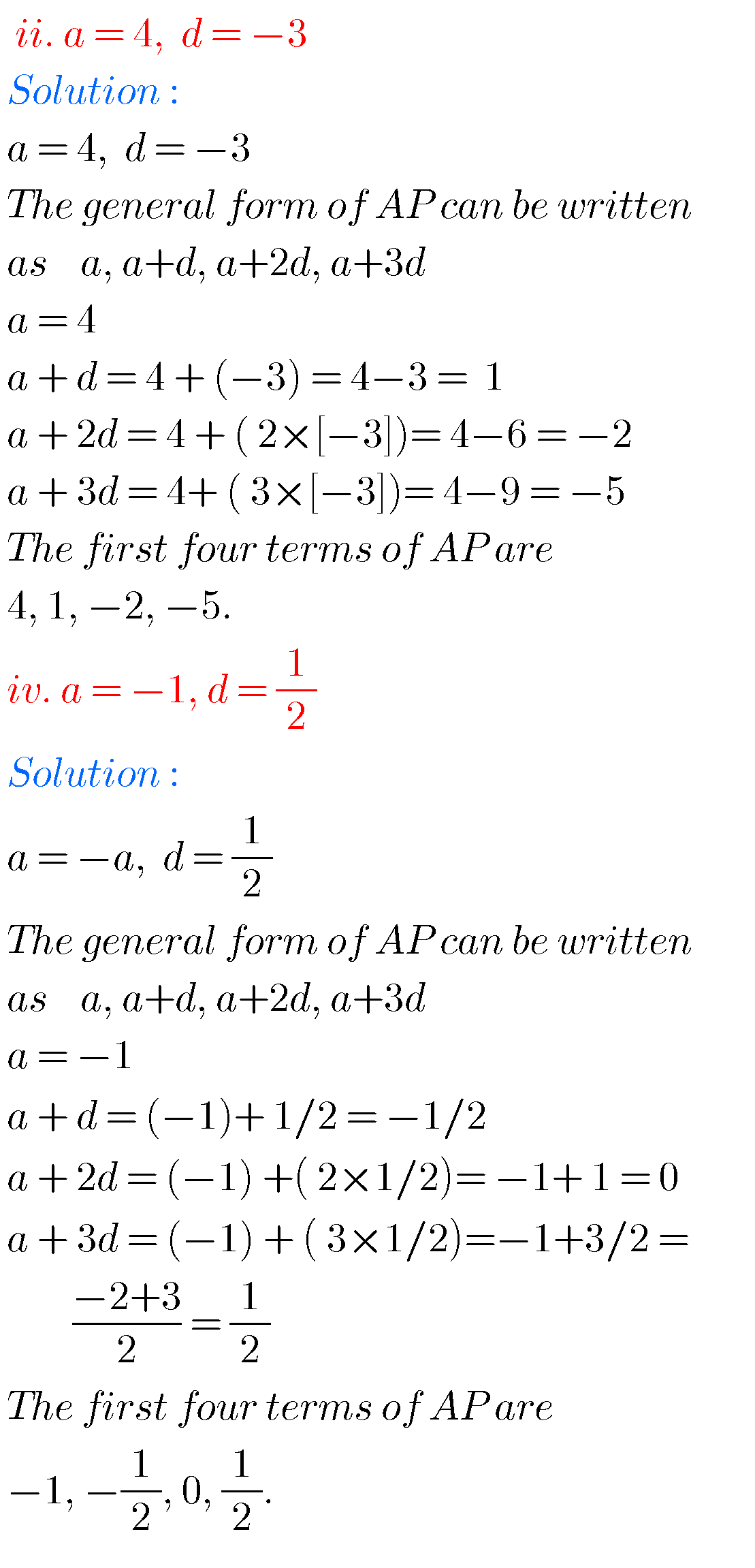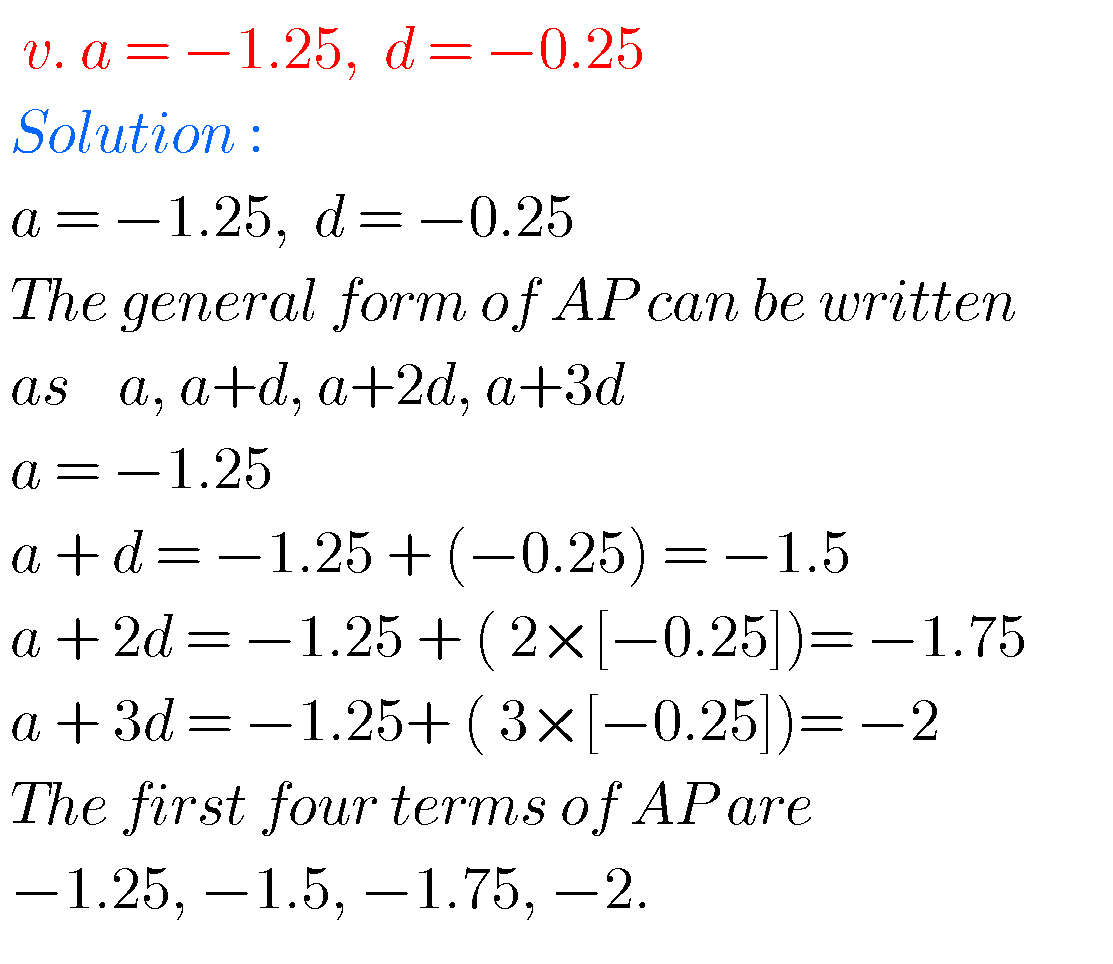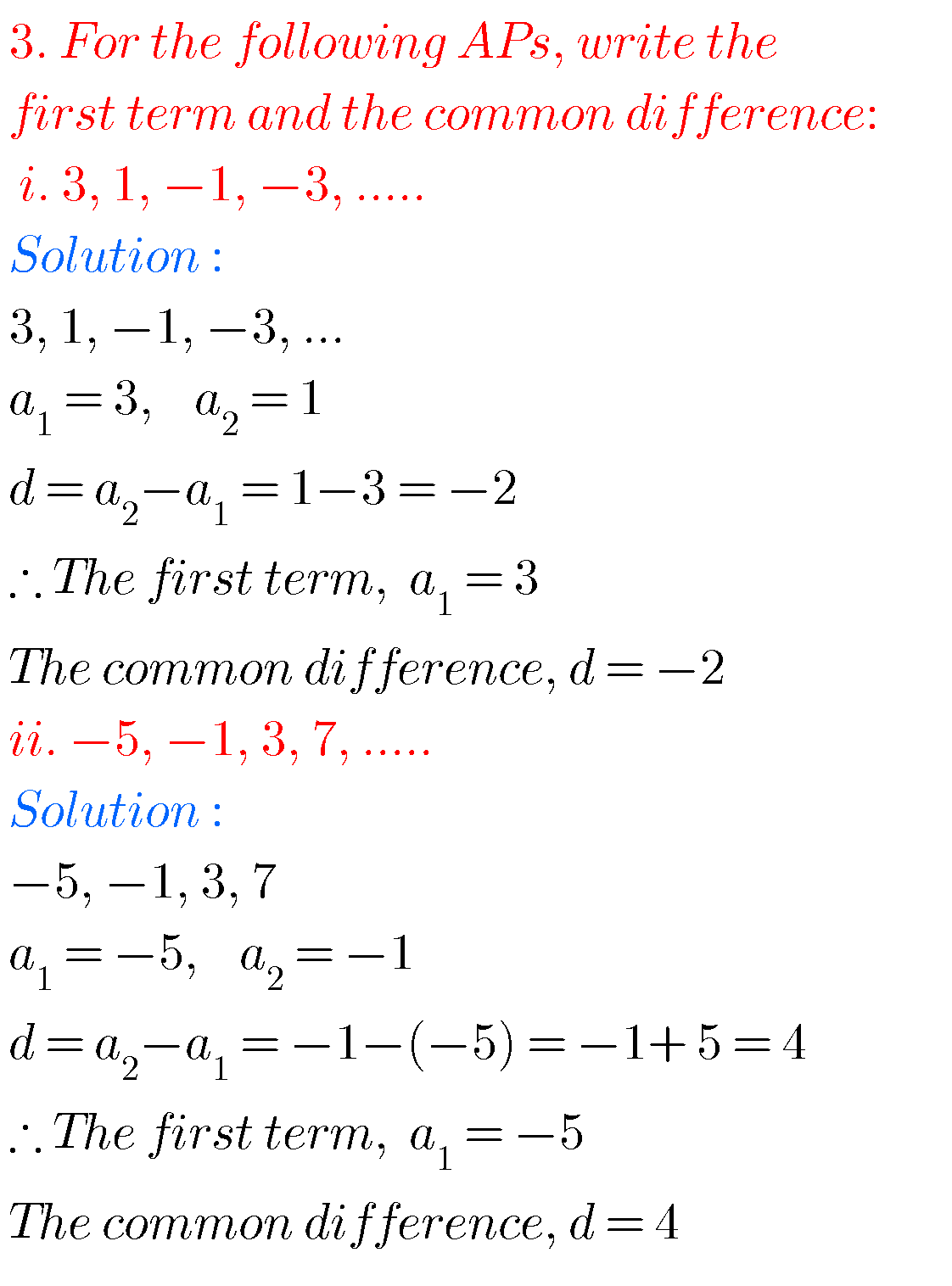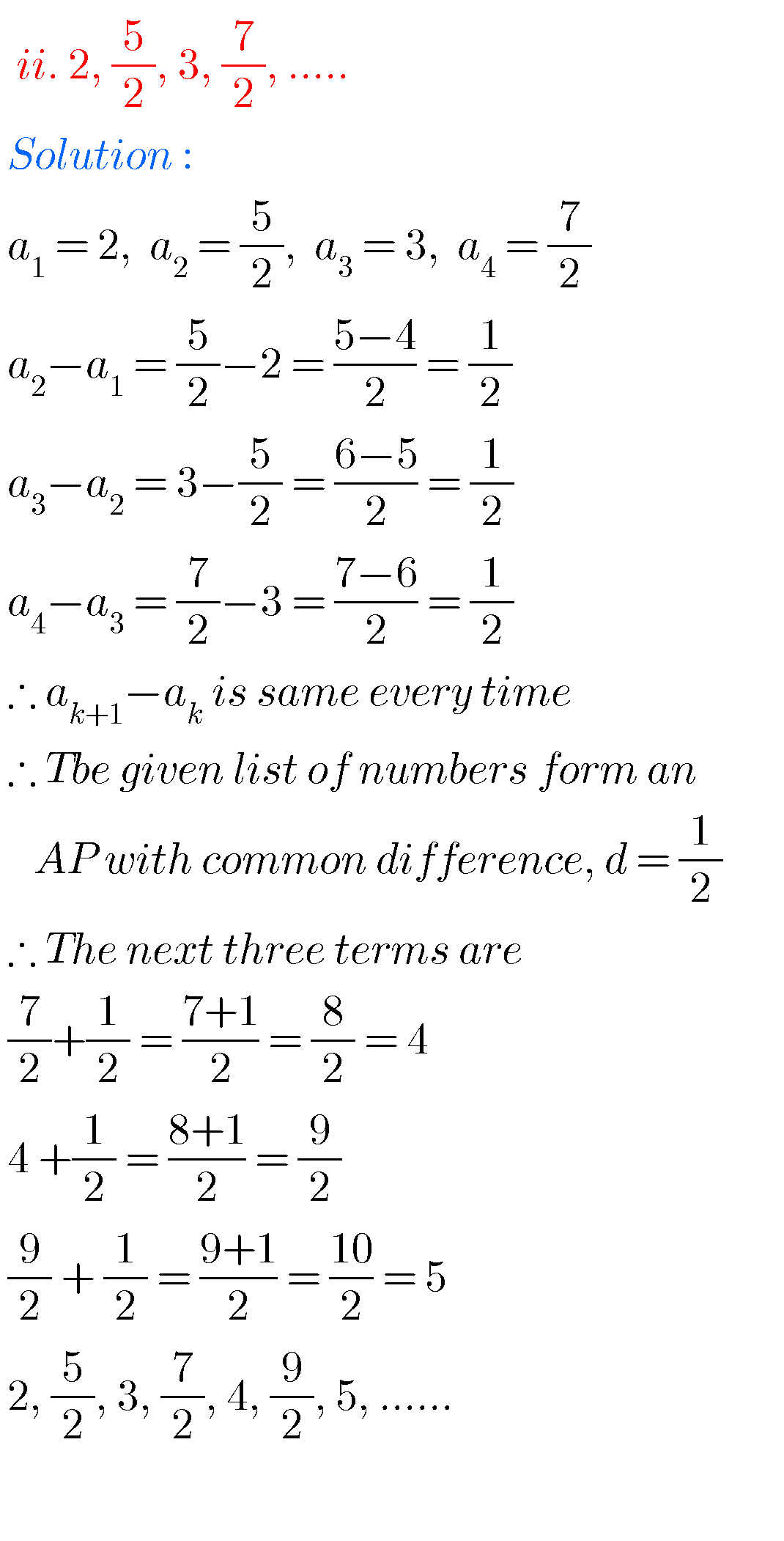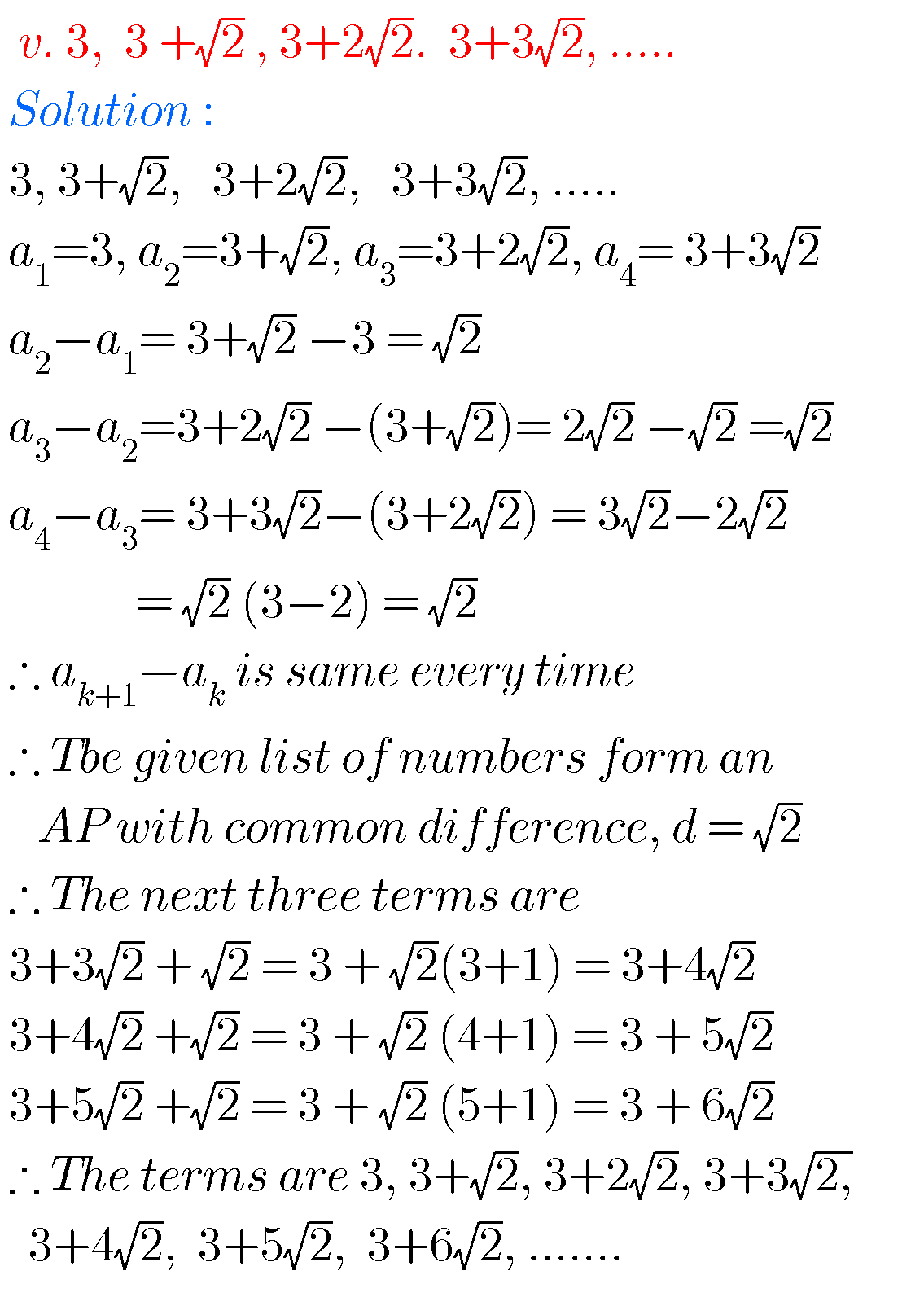##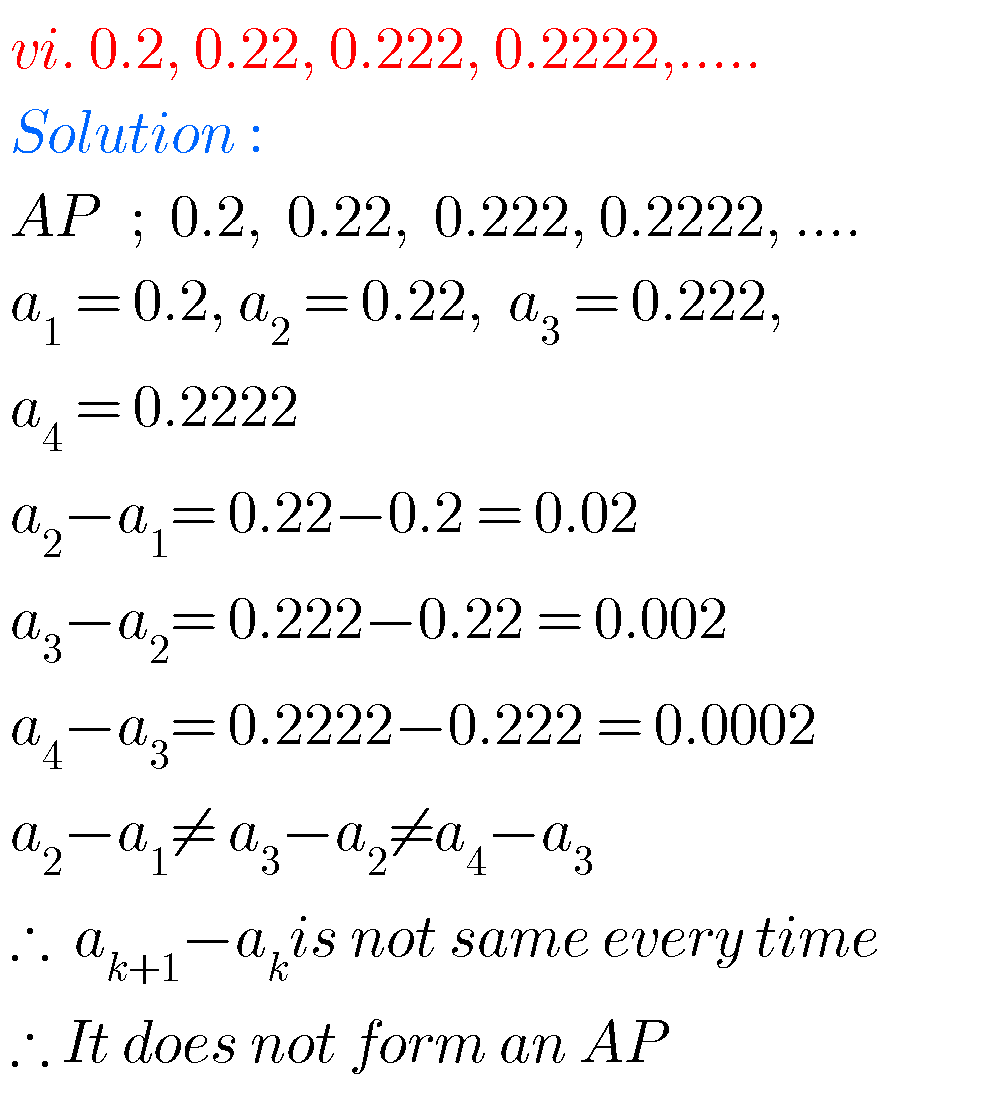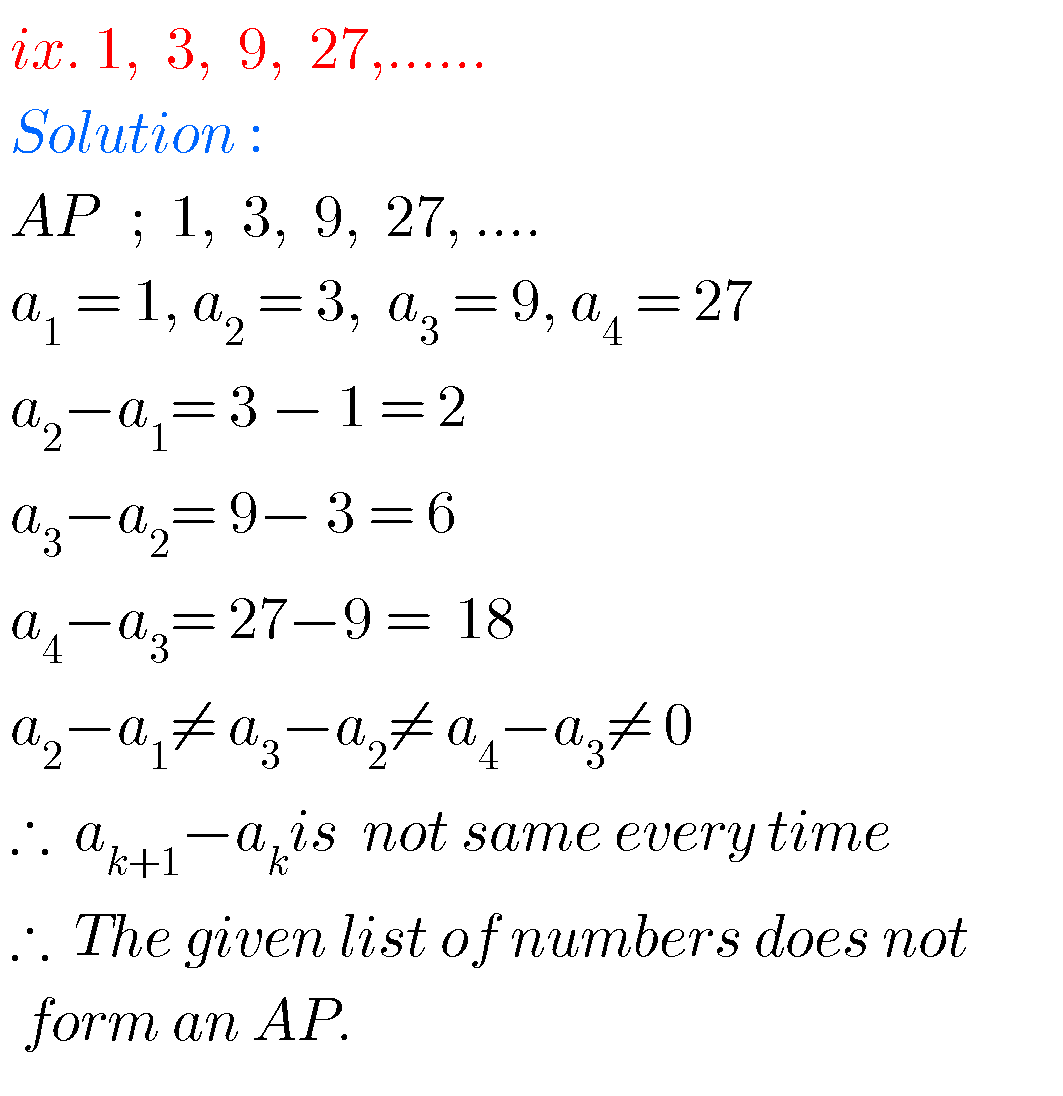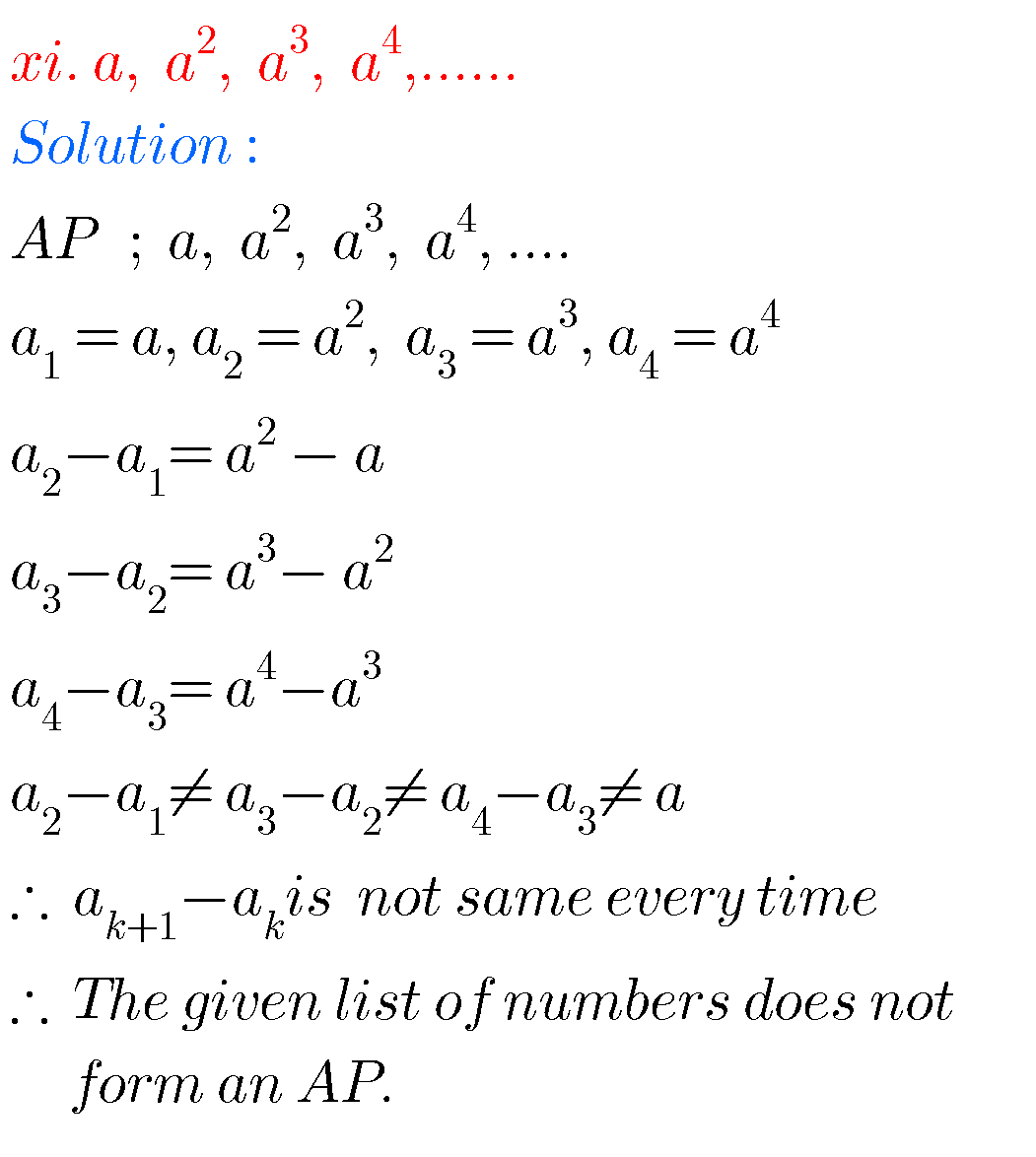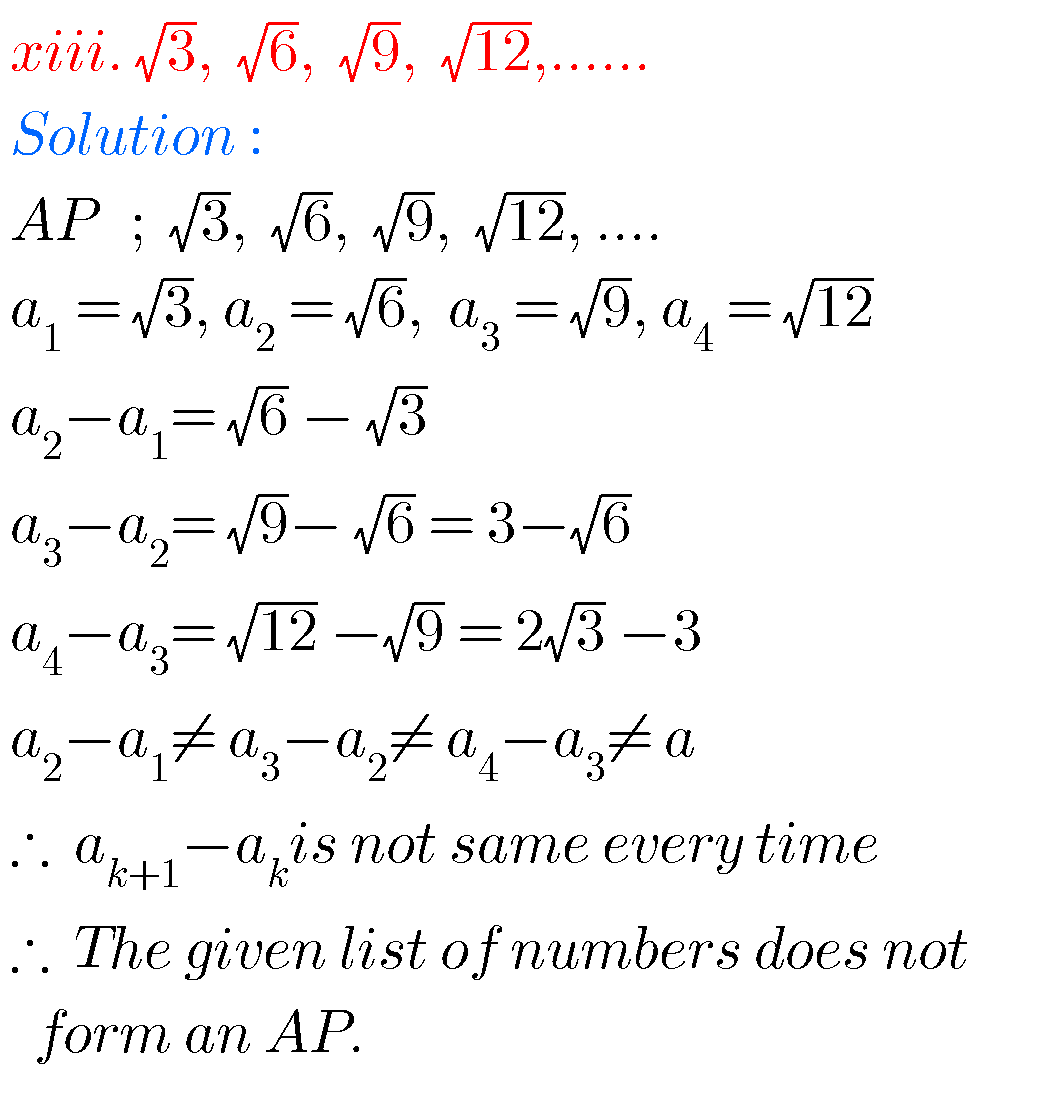## Maths solutions for Progressions class 10 SSC

Exercise 6.2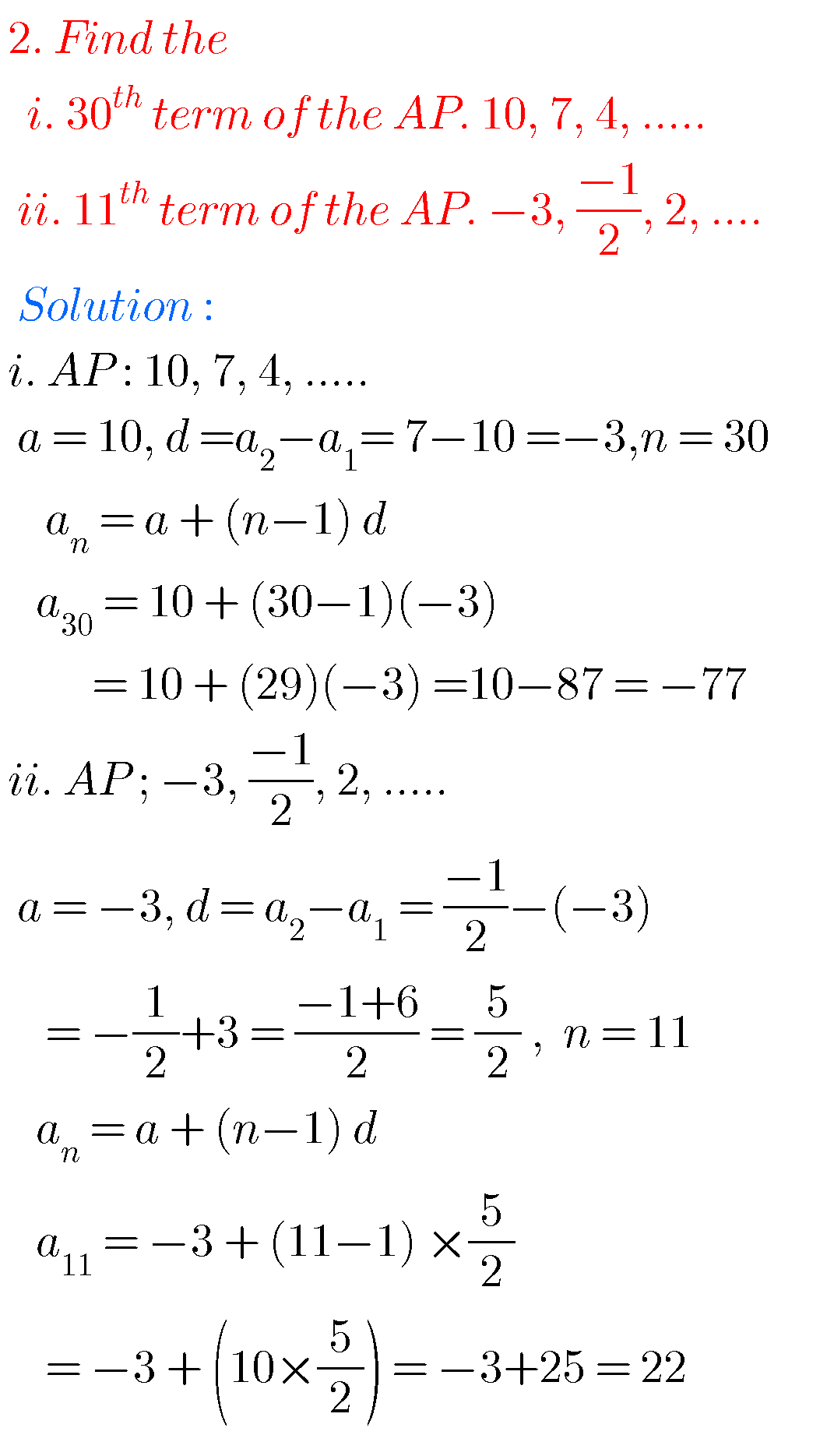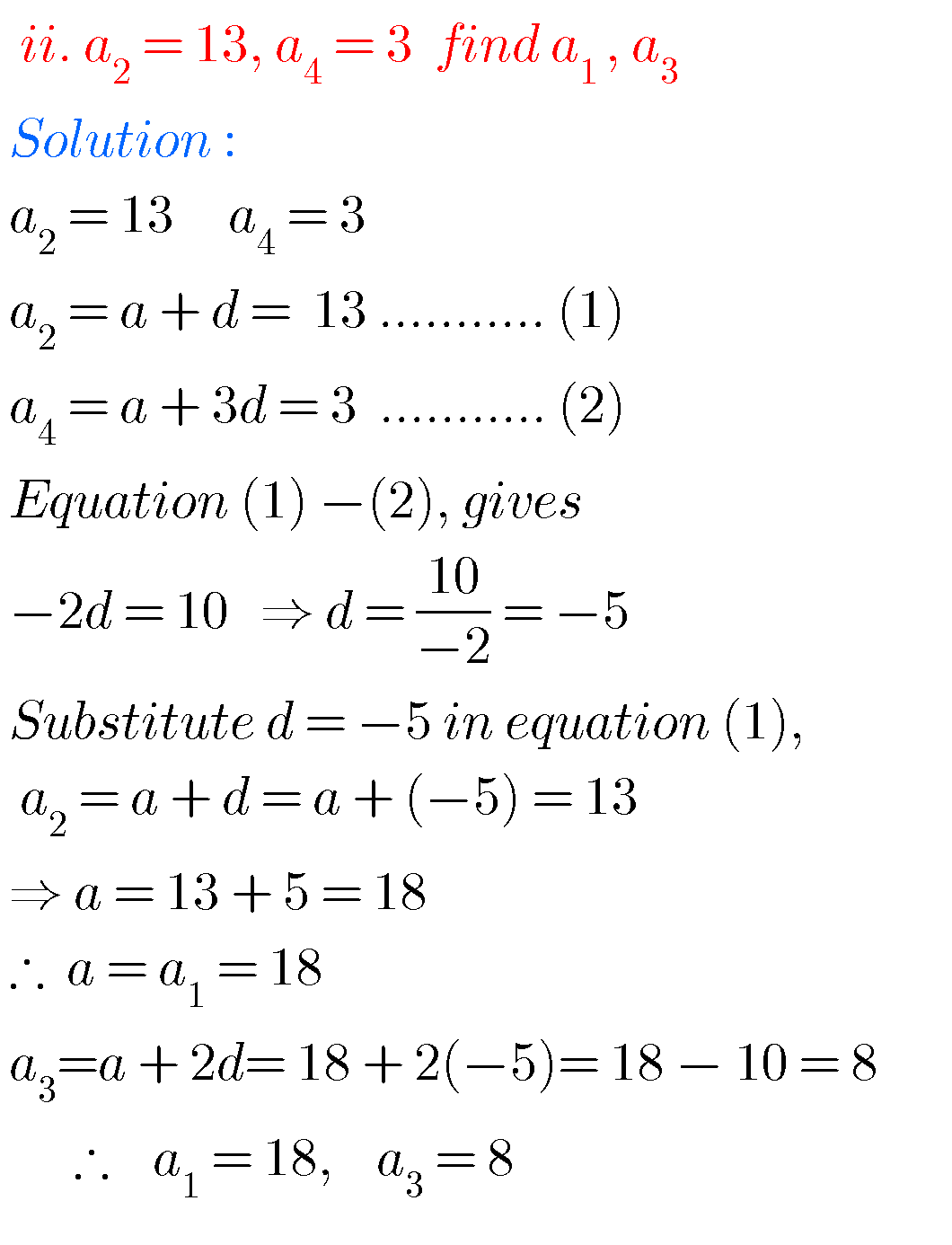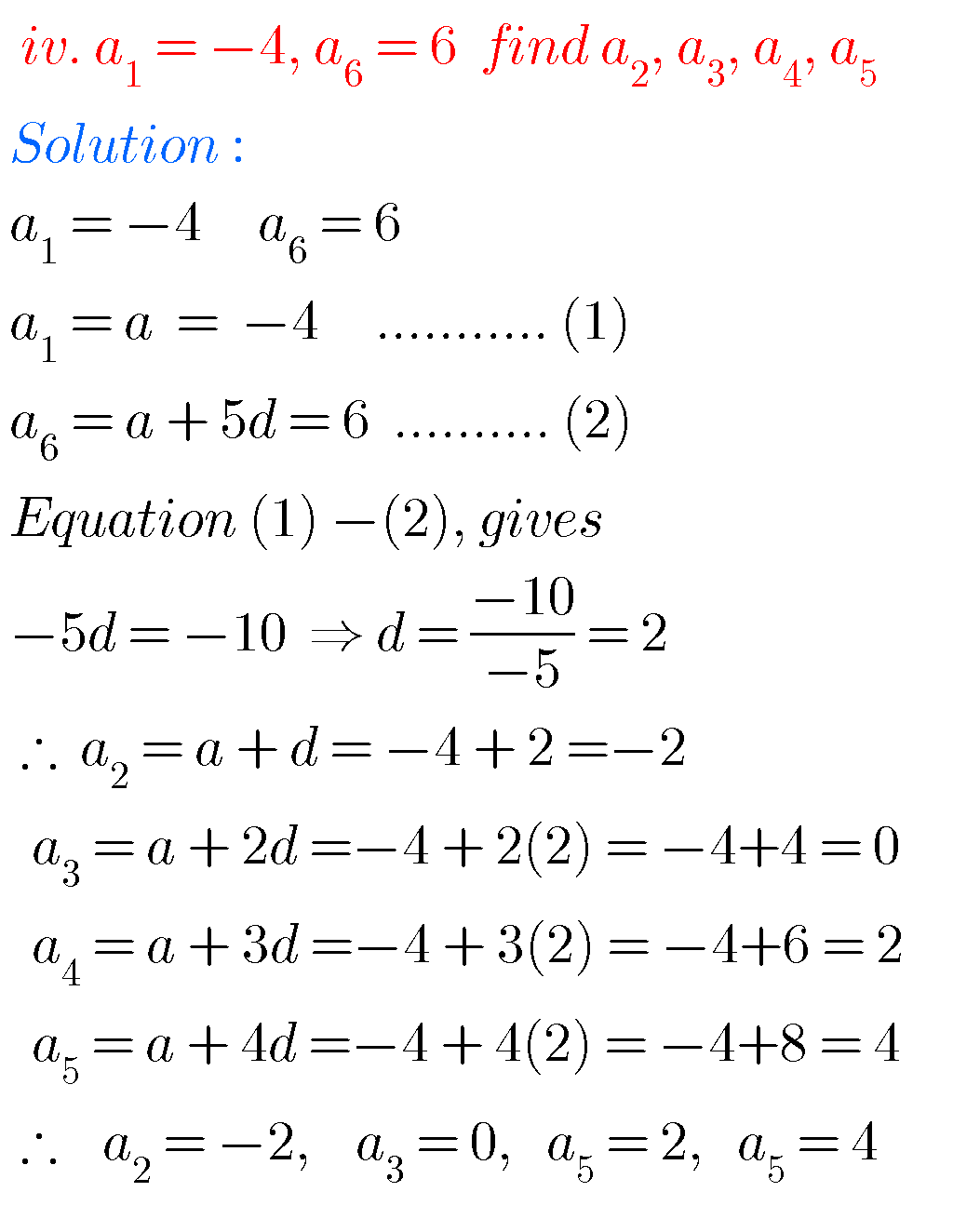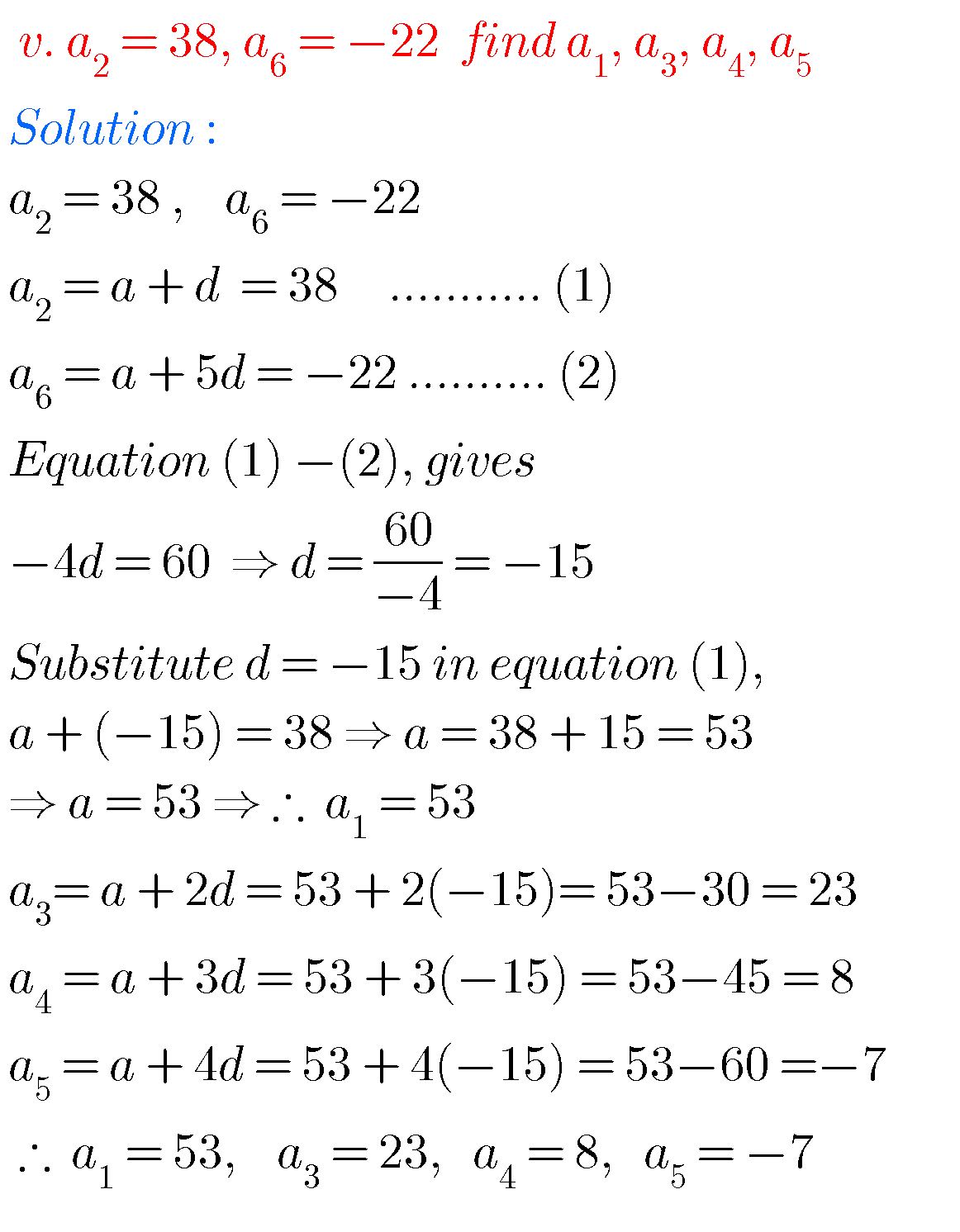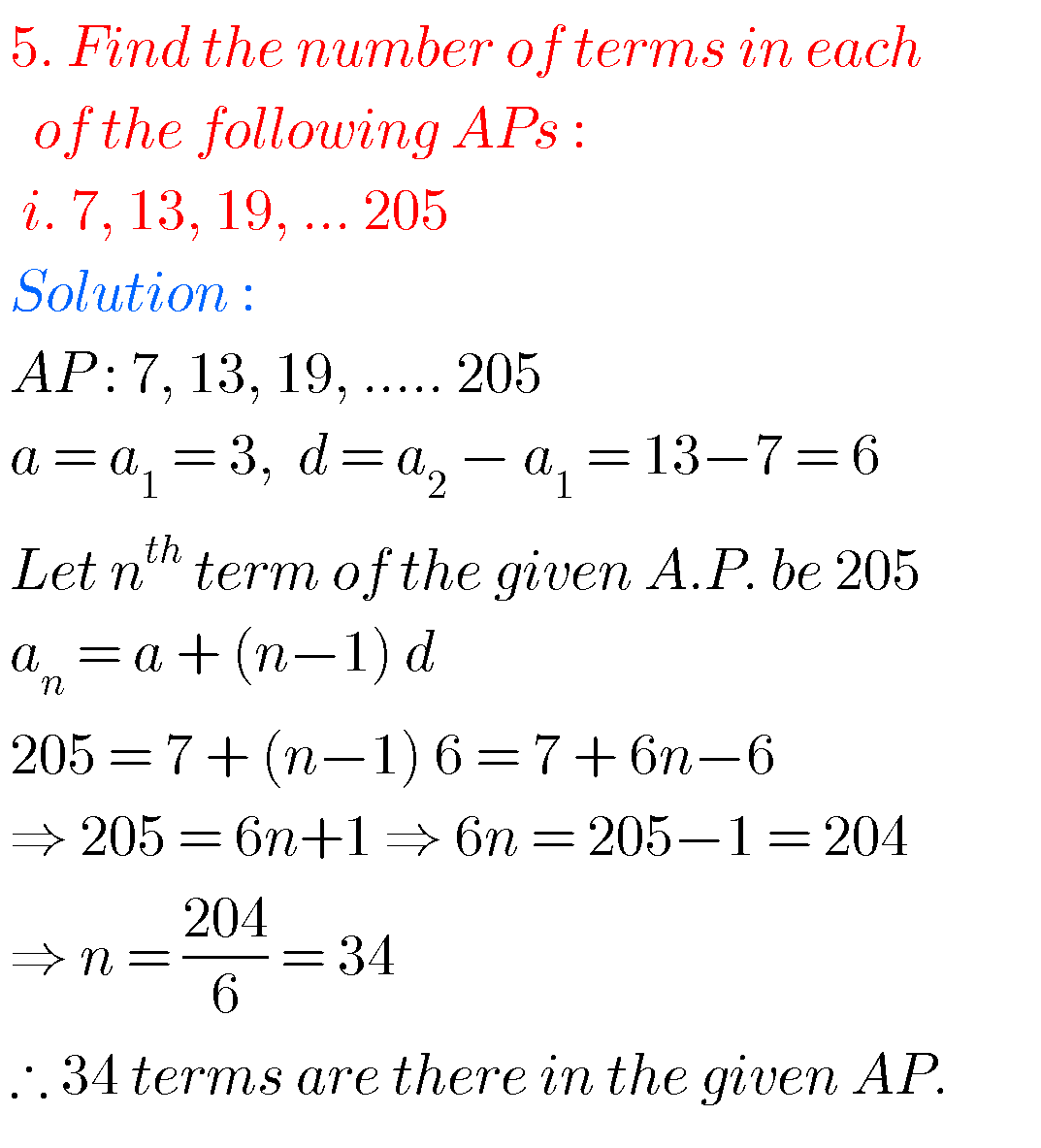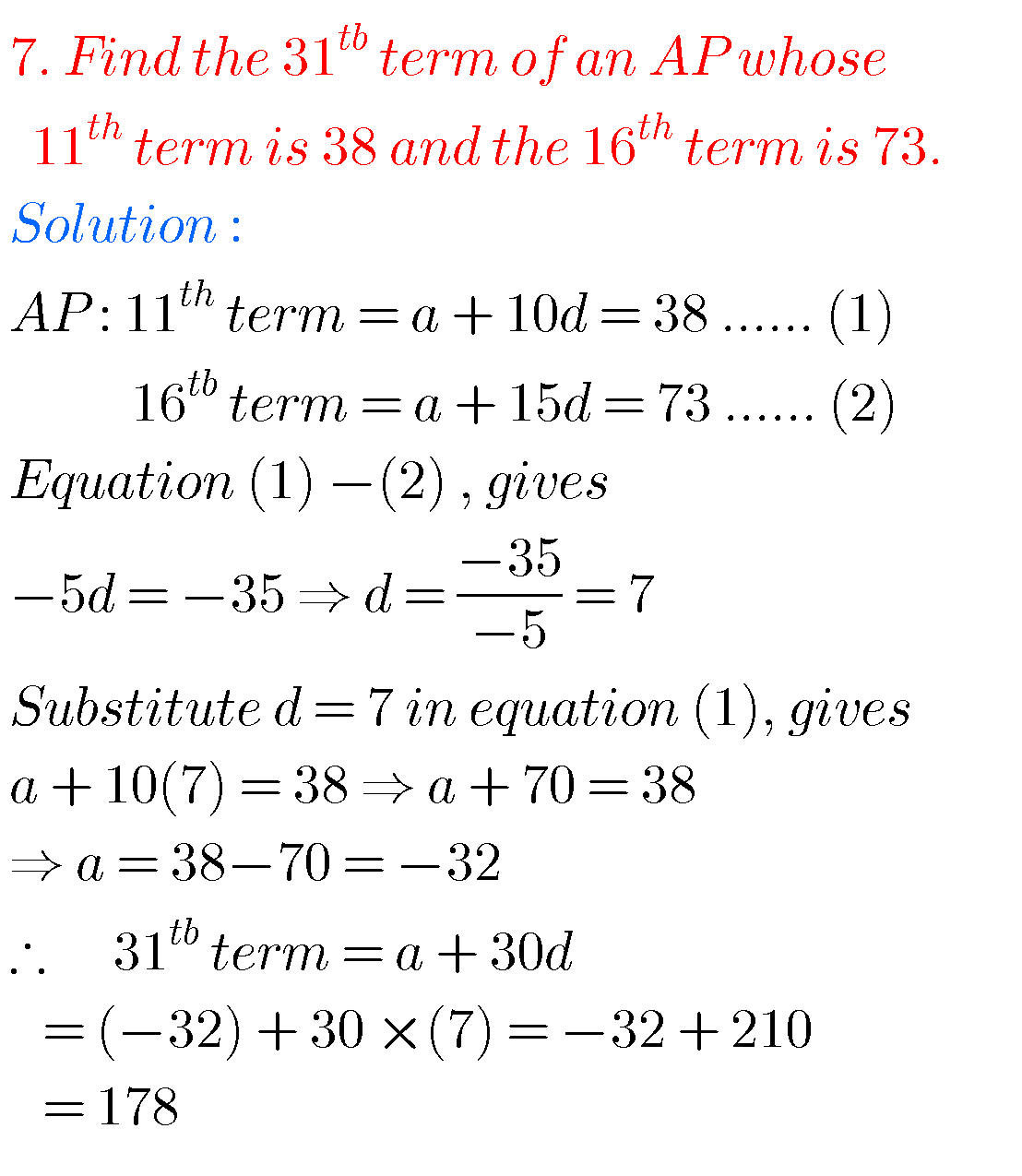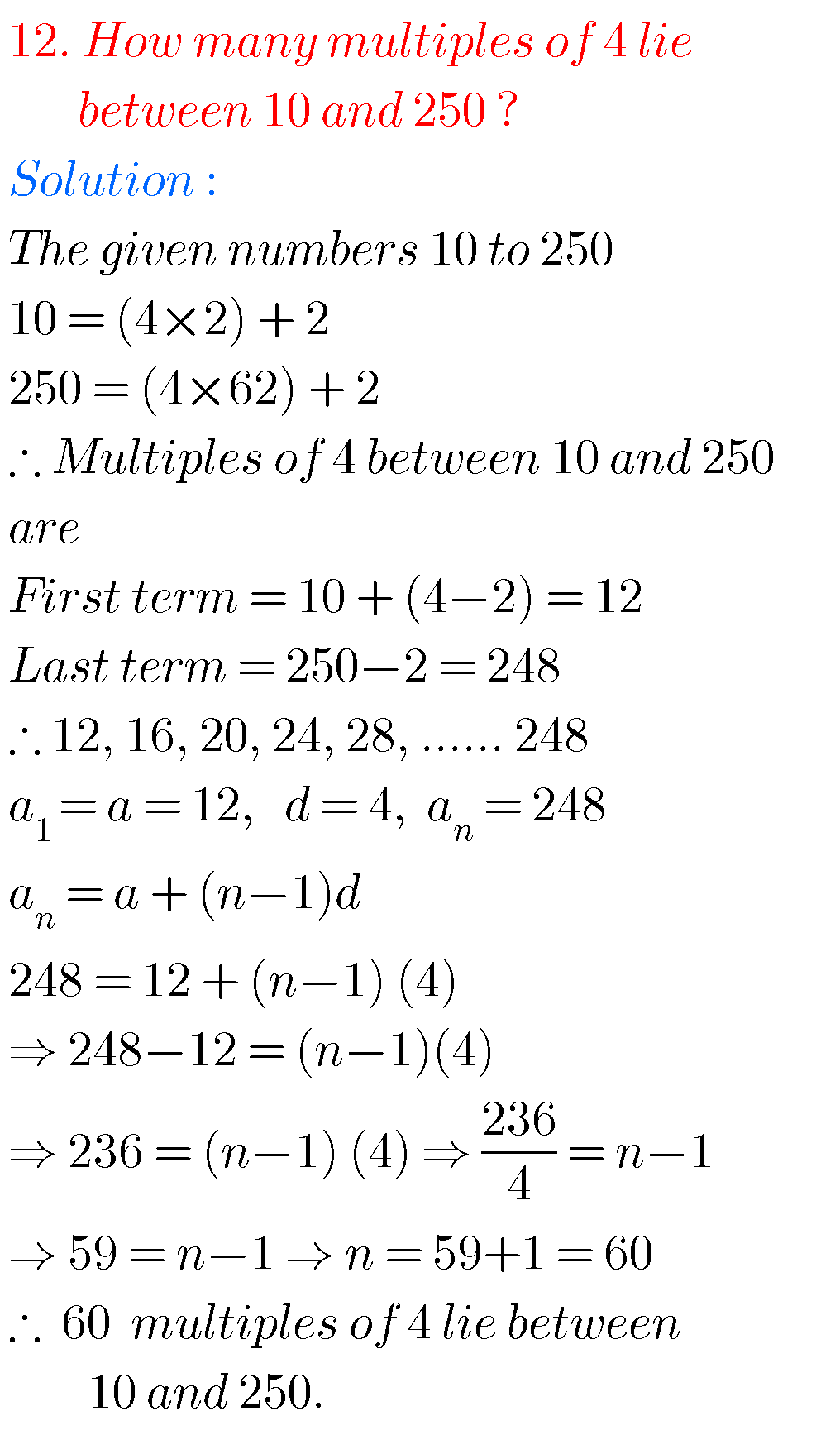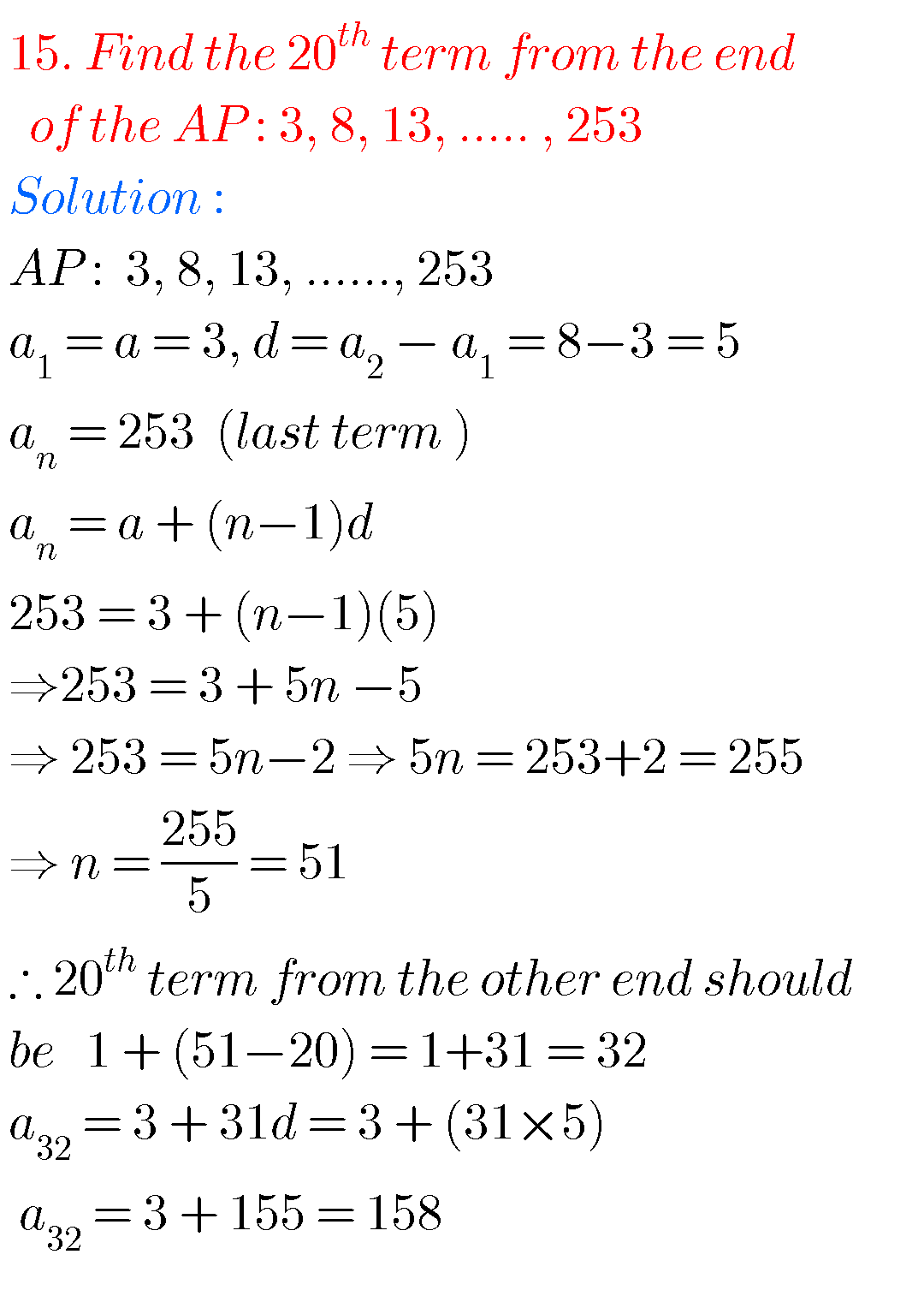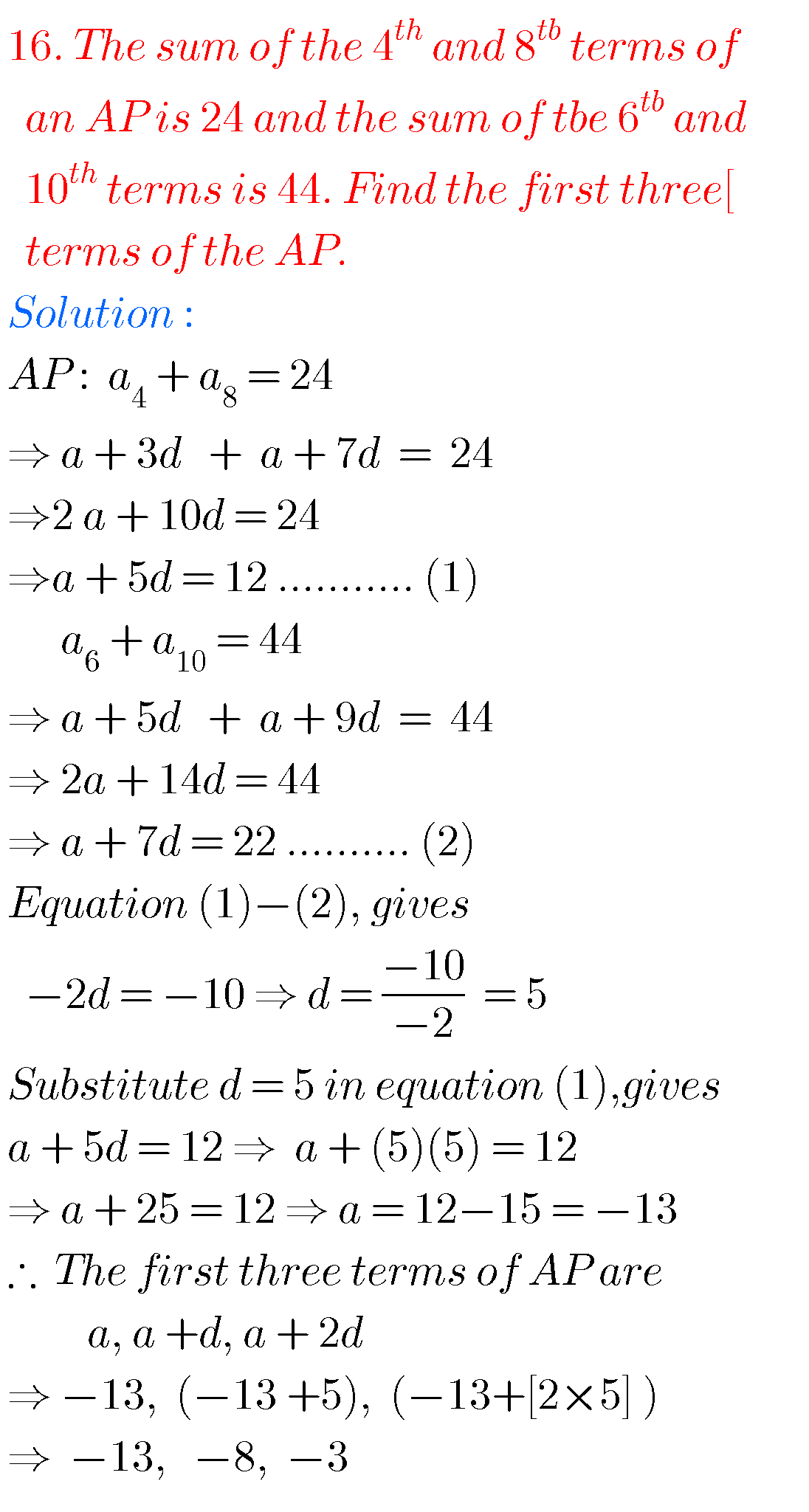## 10th class maths Progressions solutions ssc

Exercise 6.3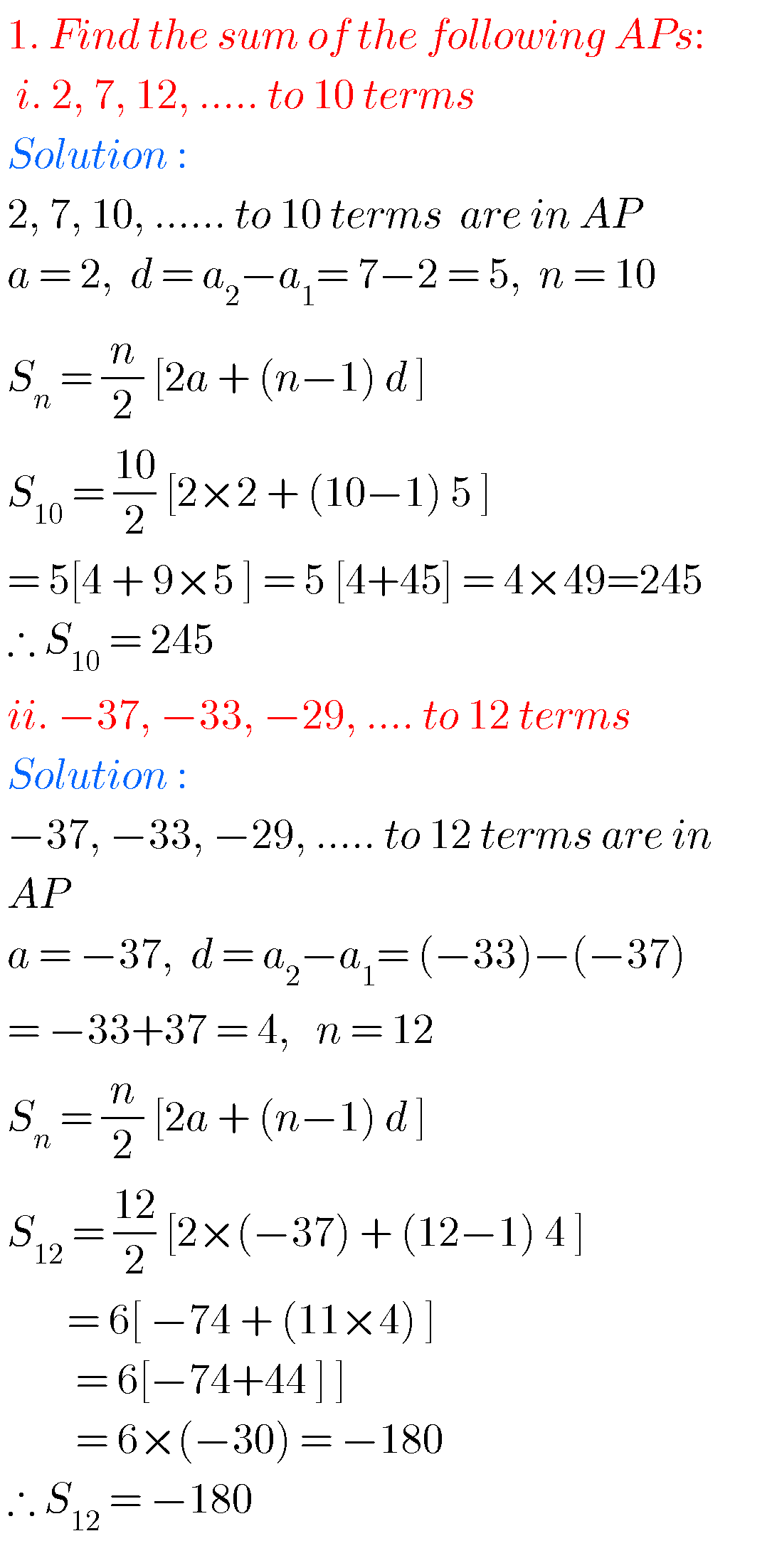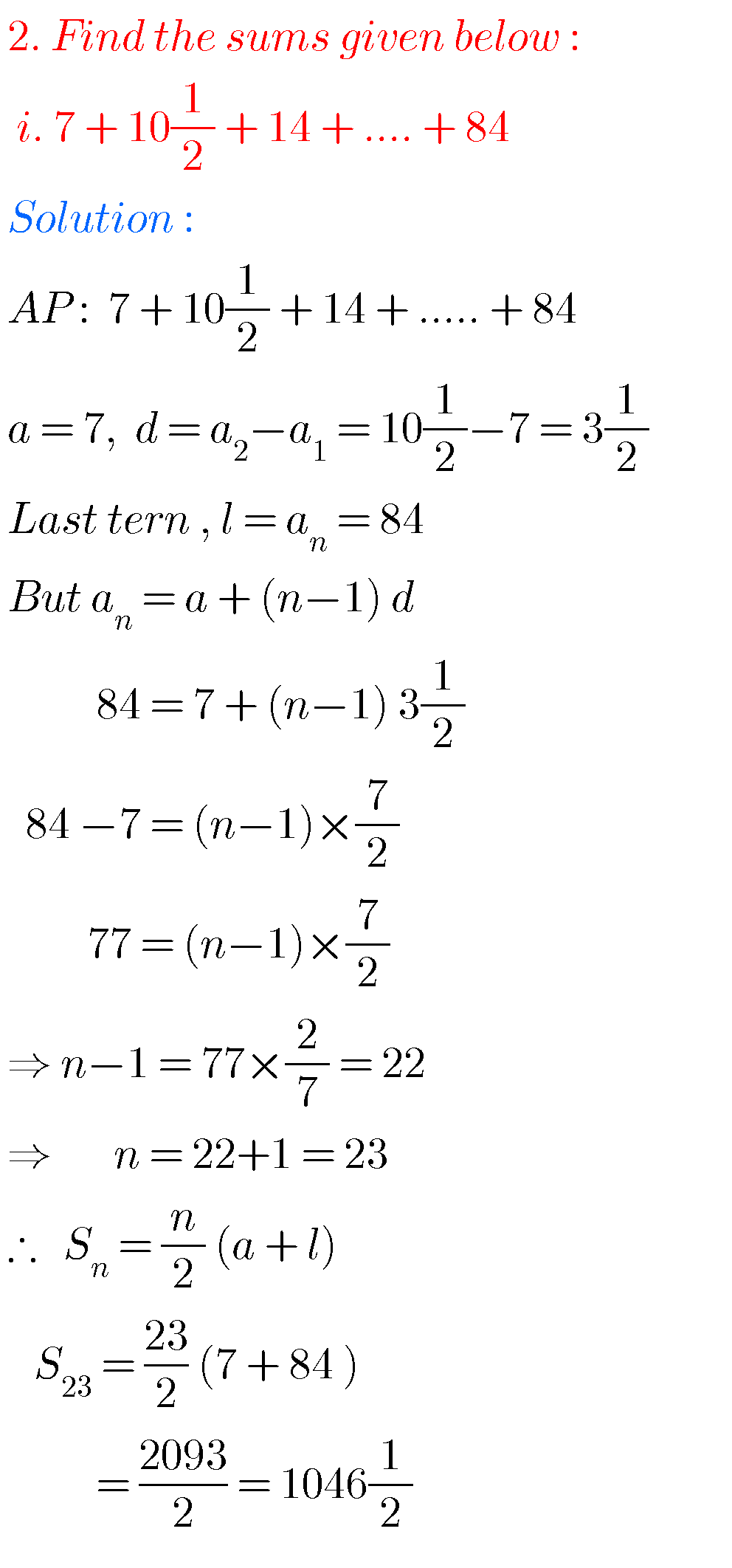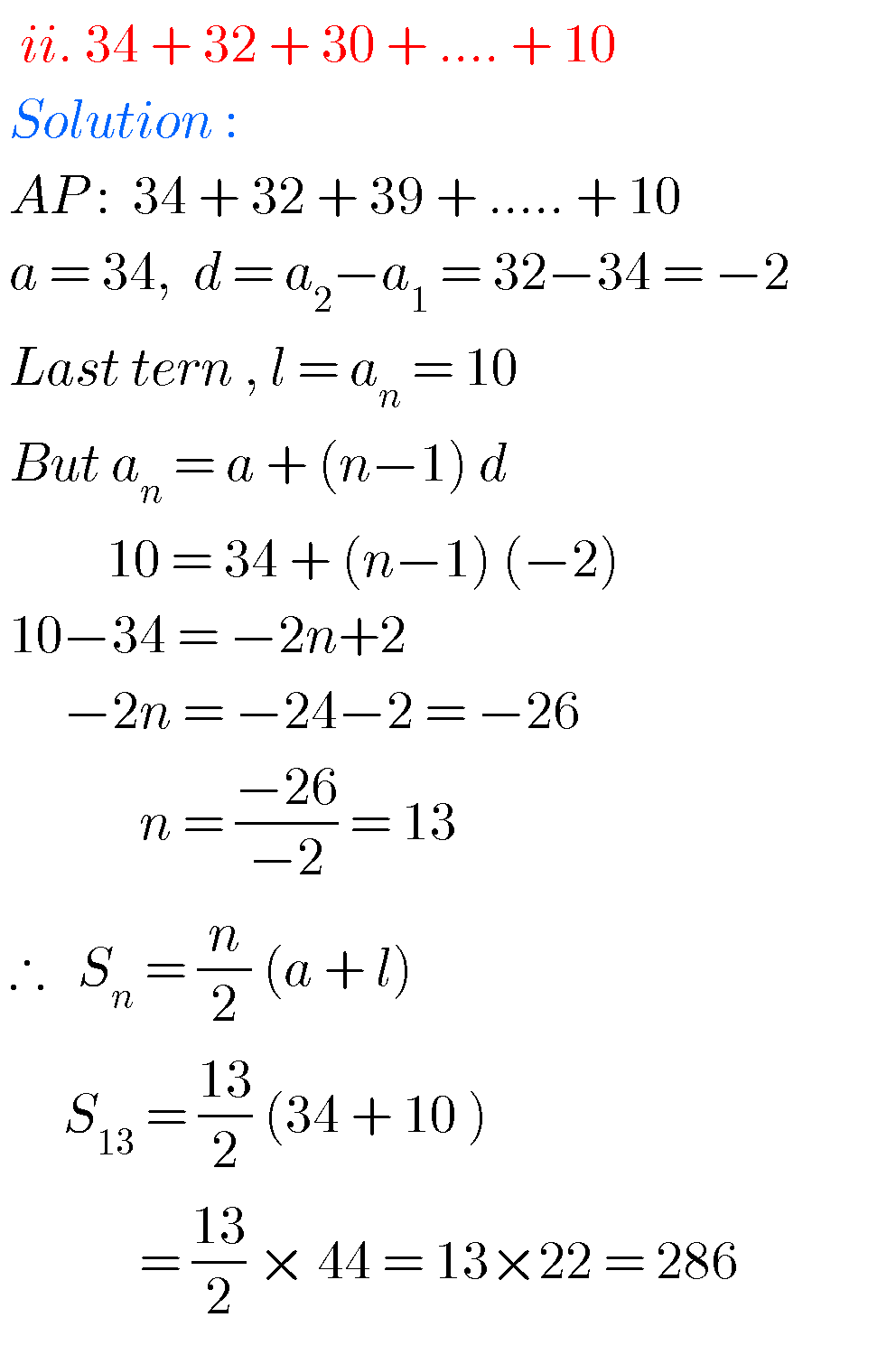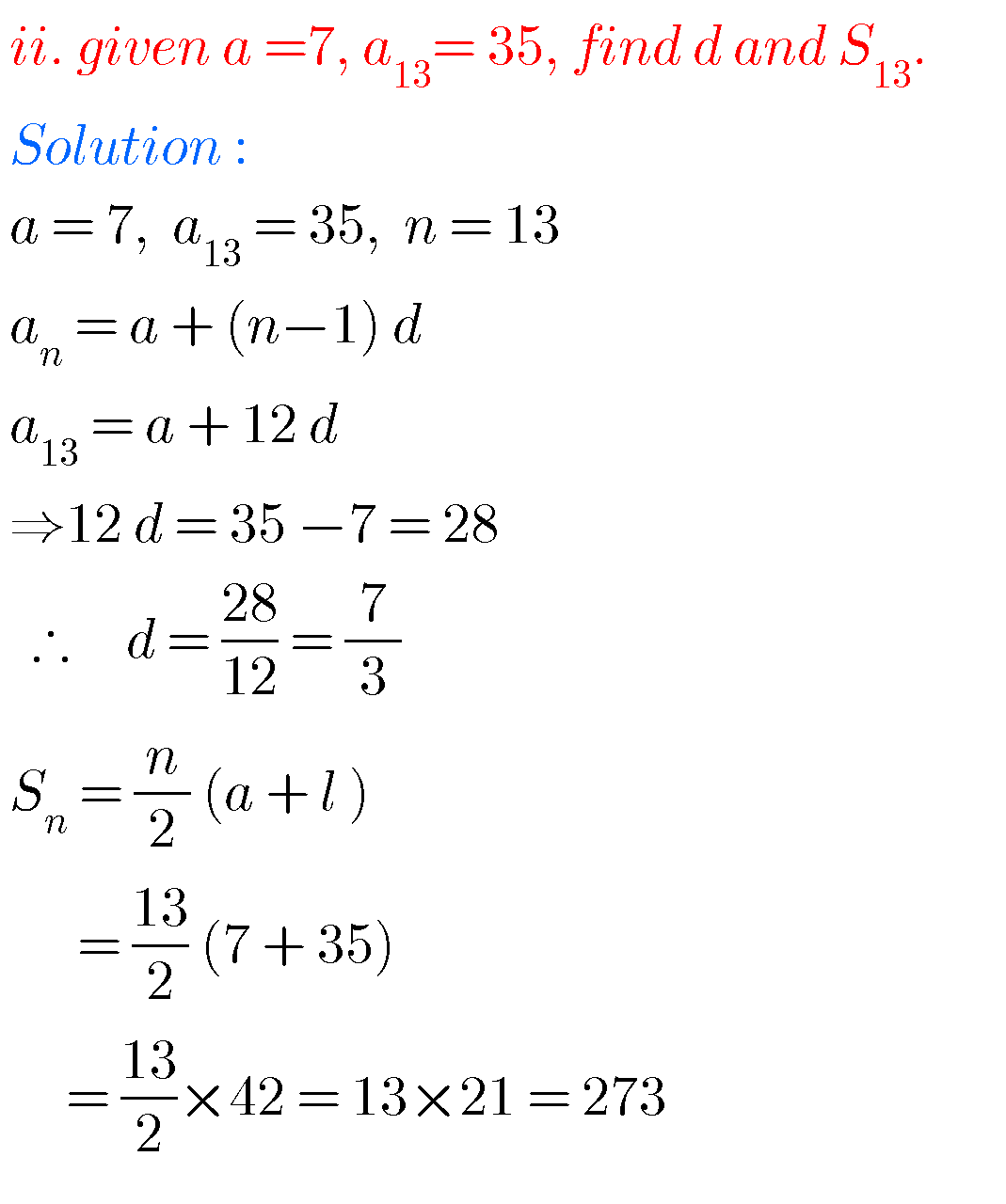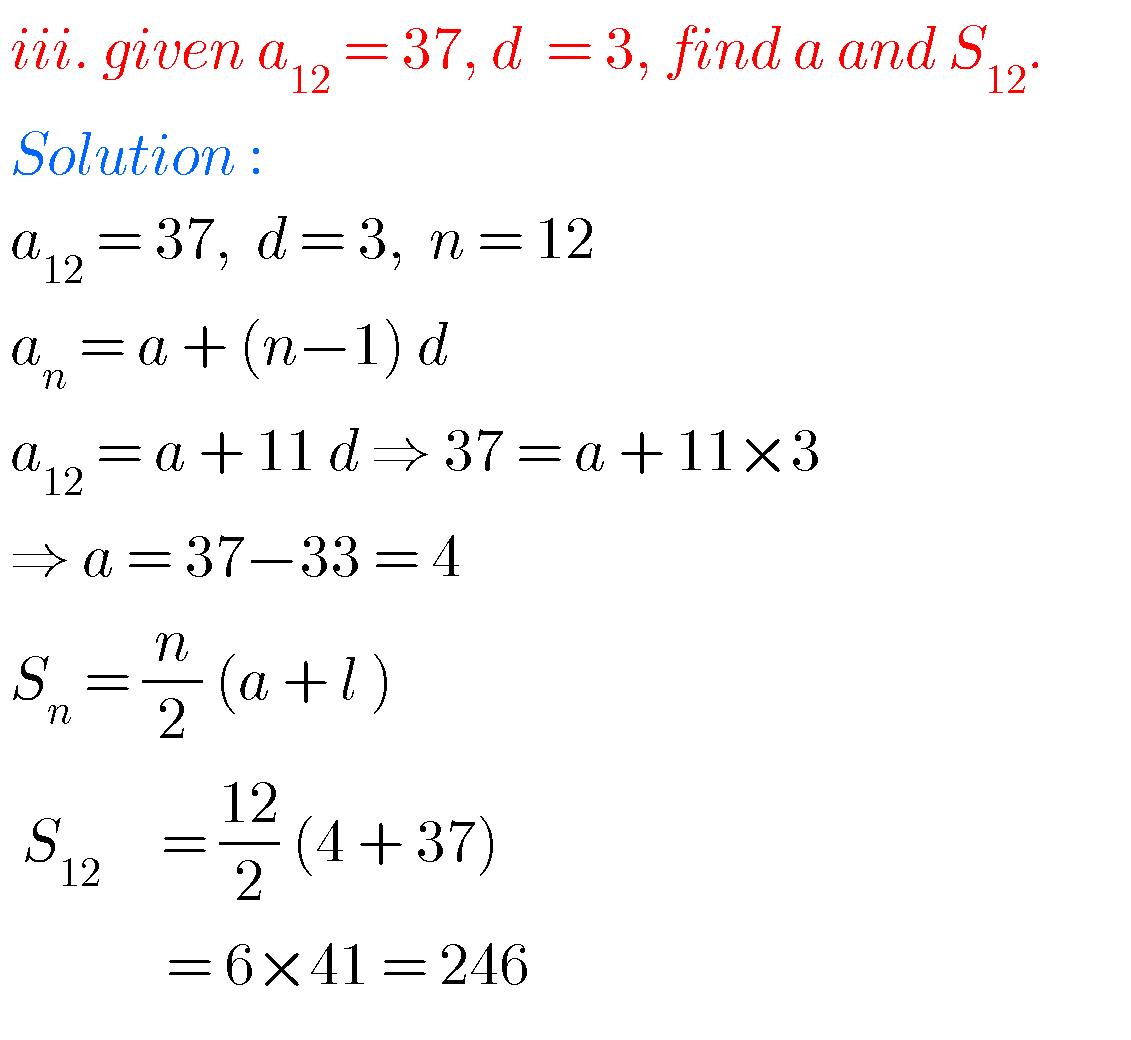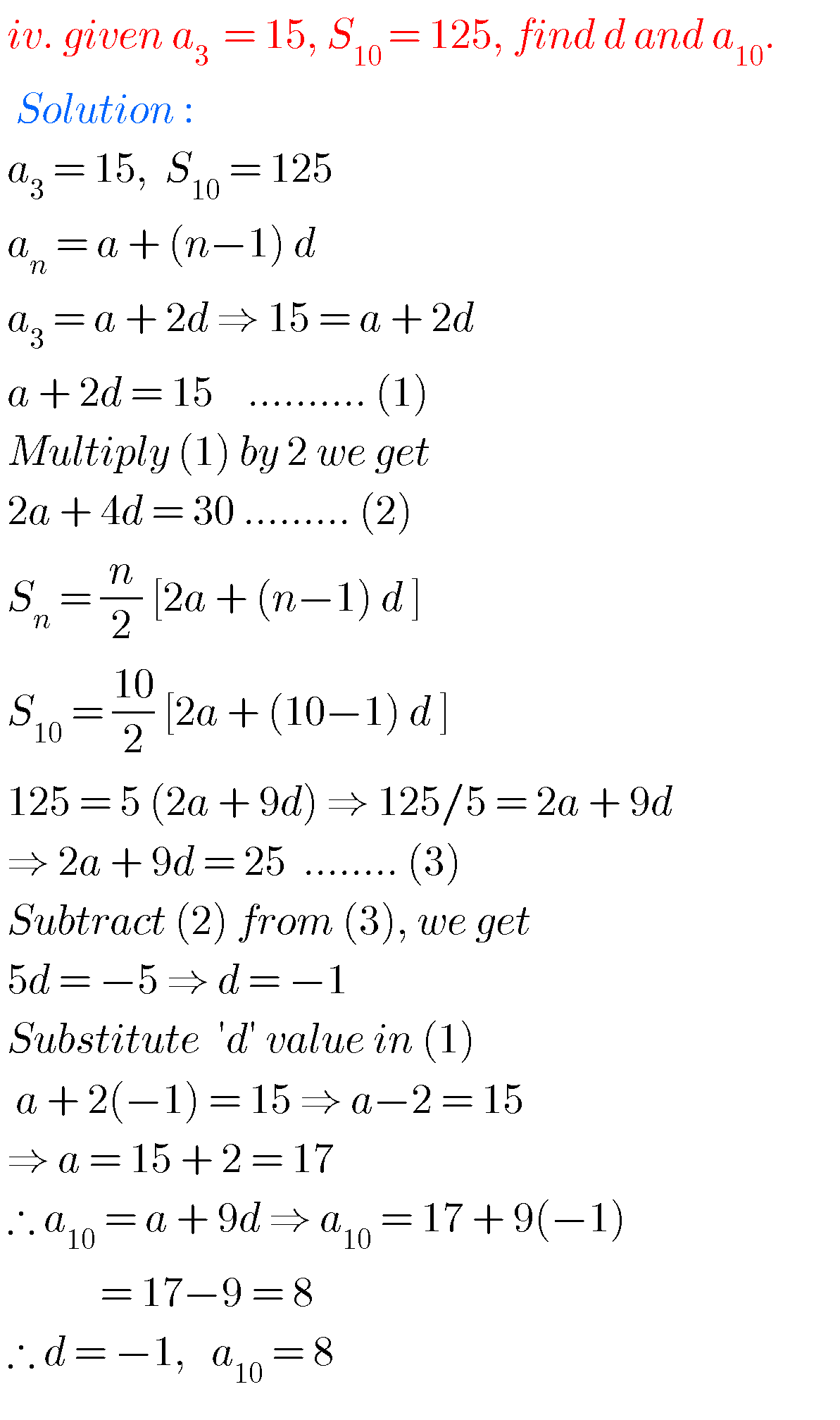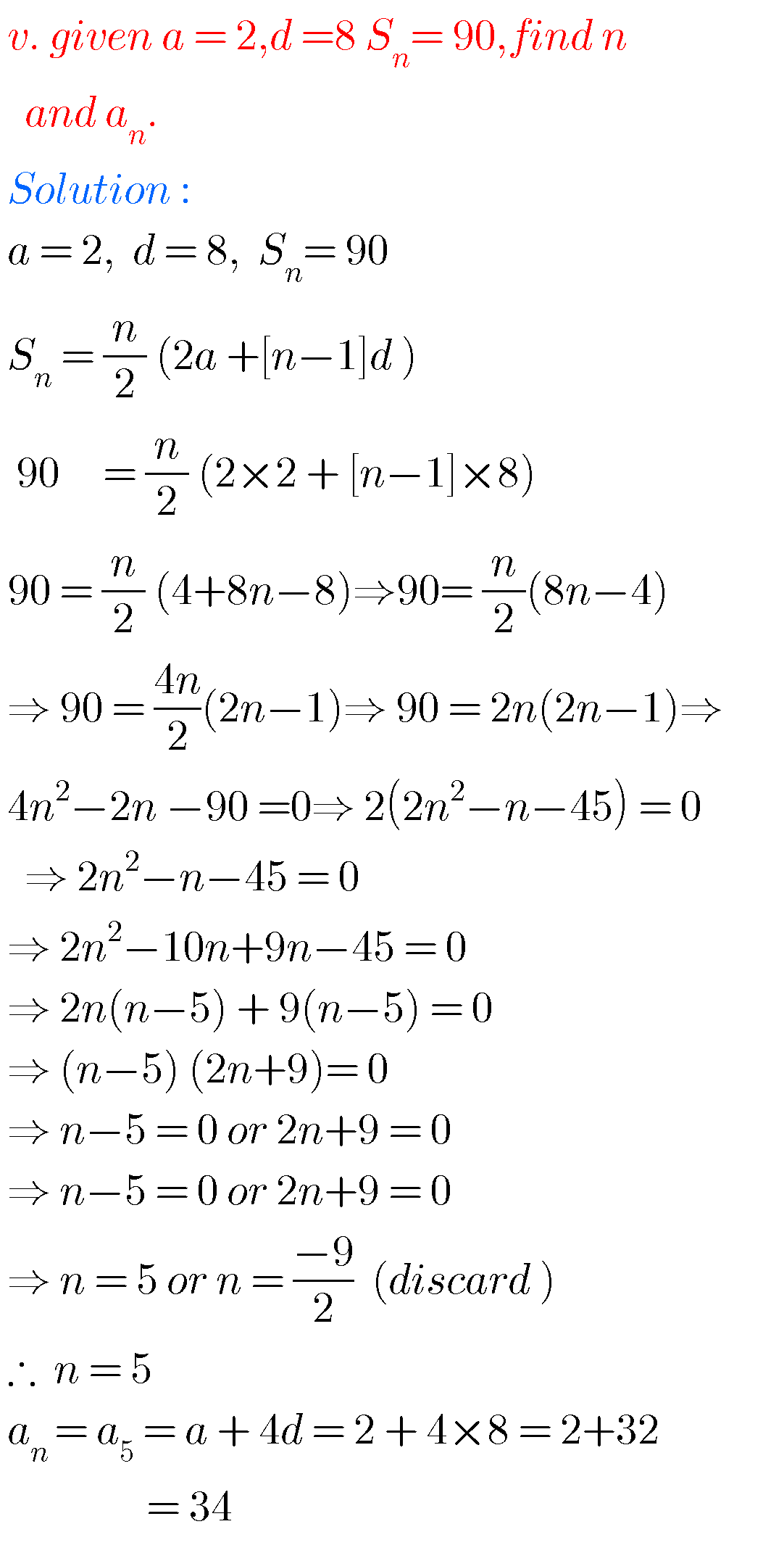###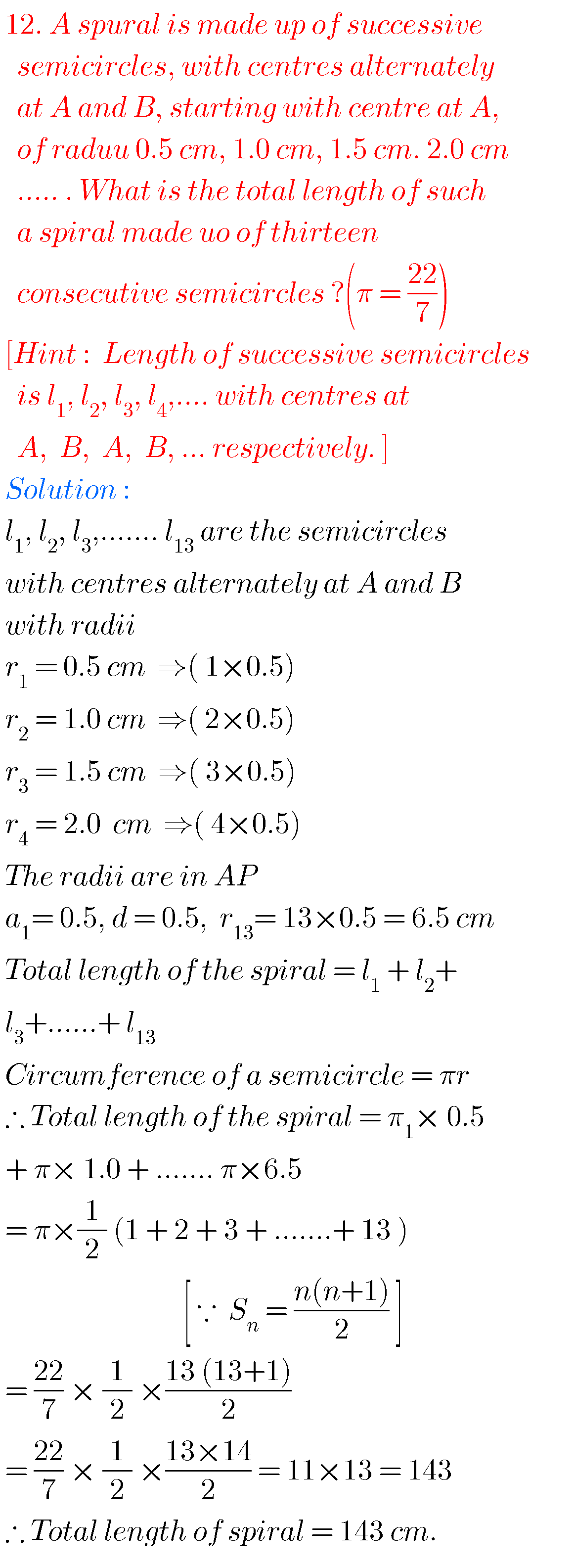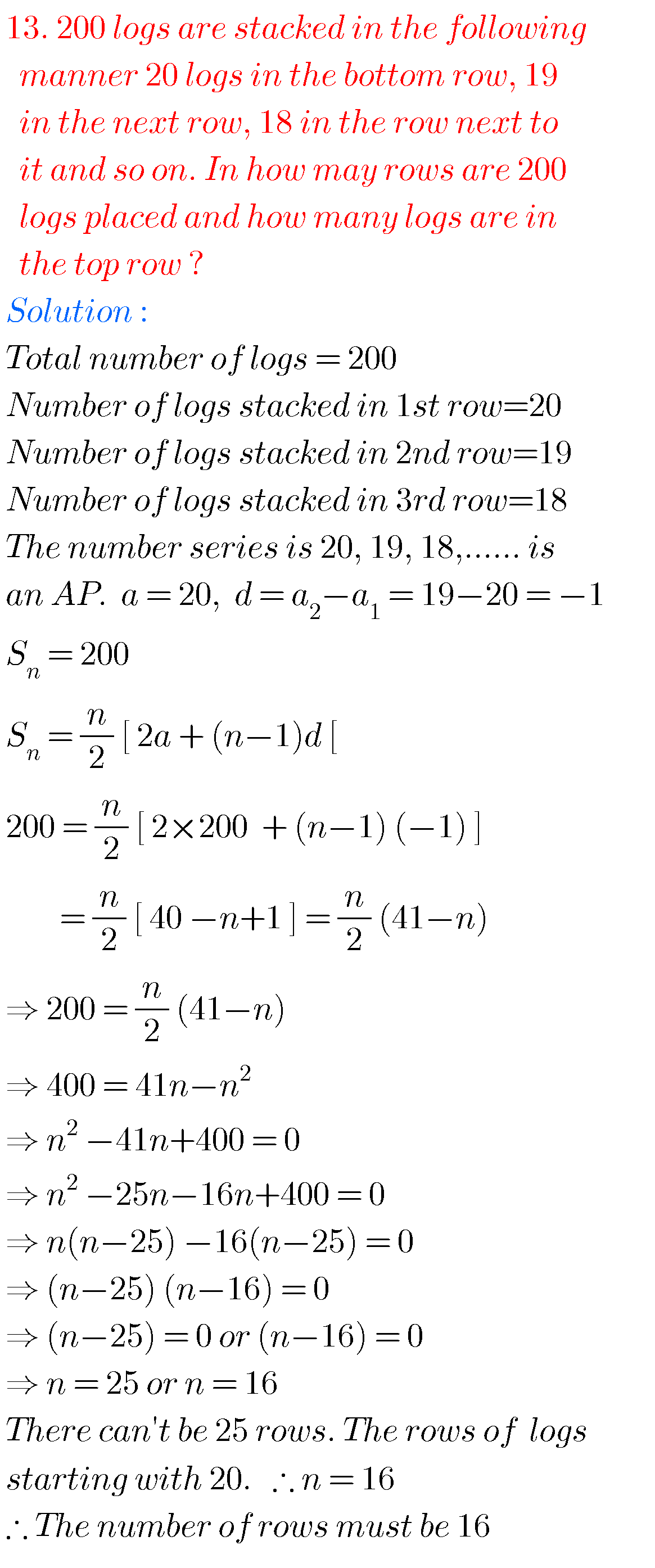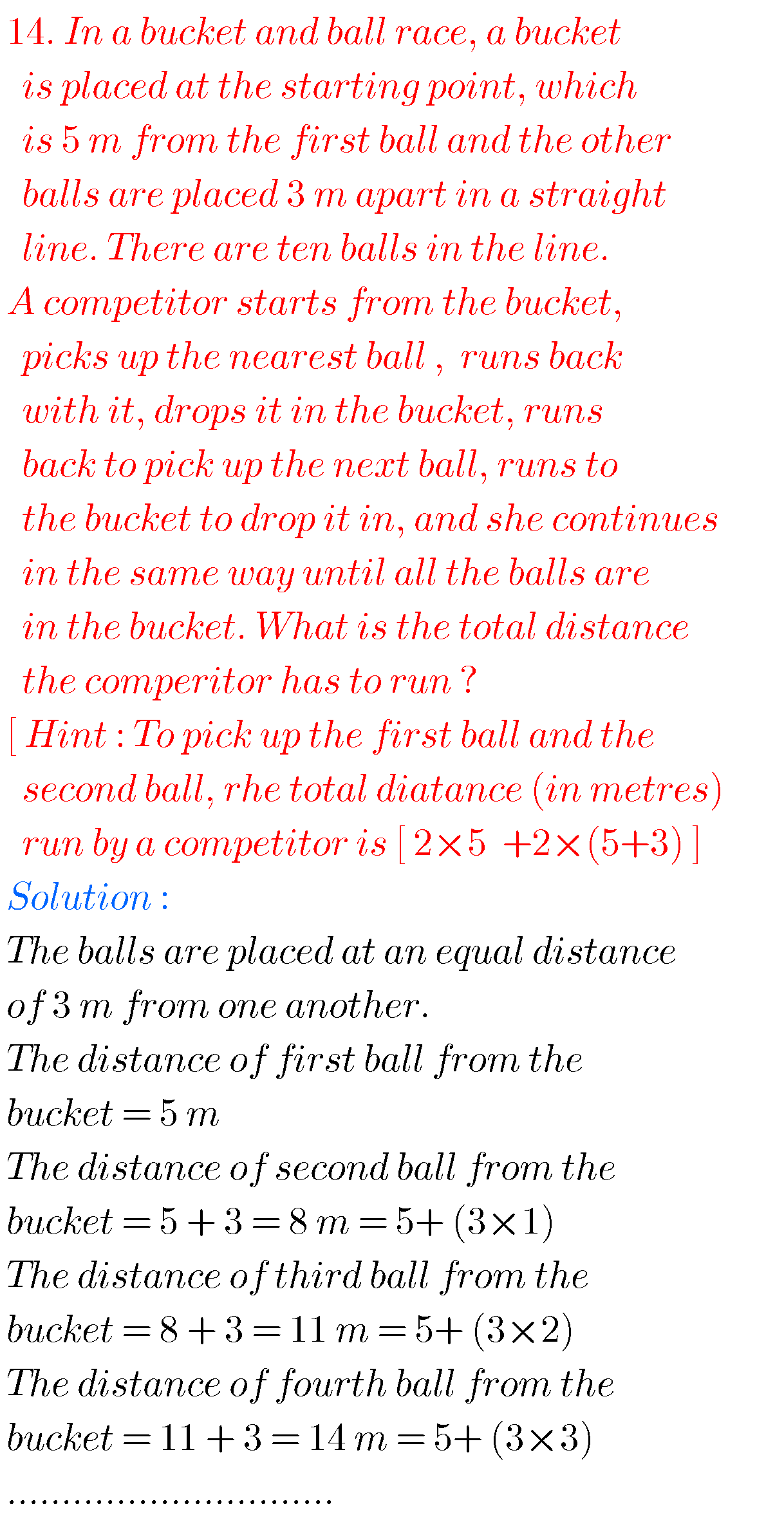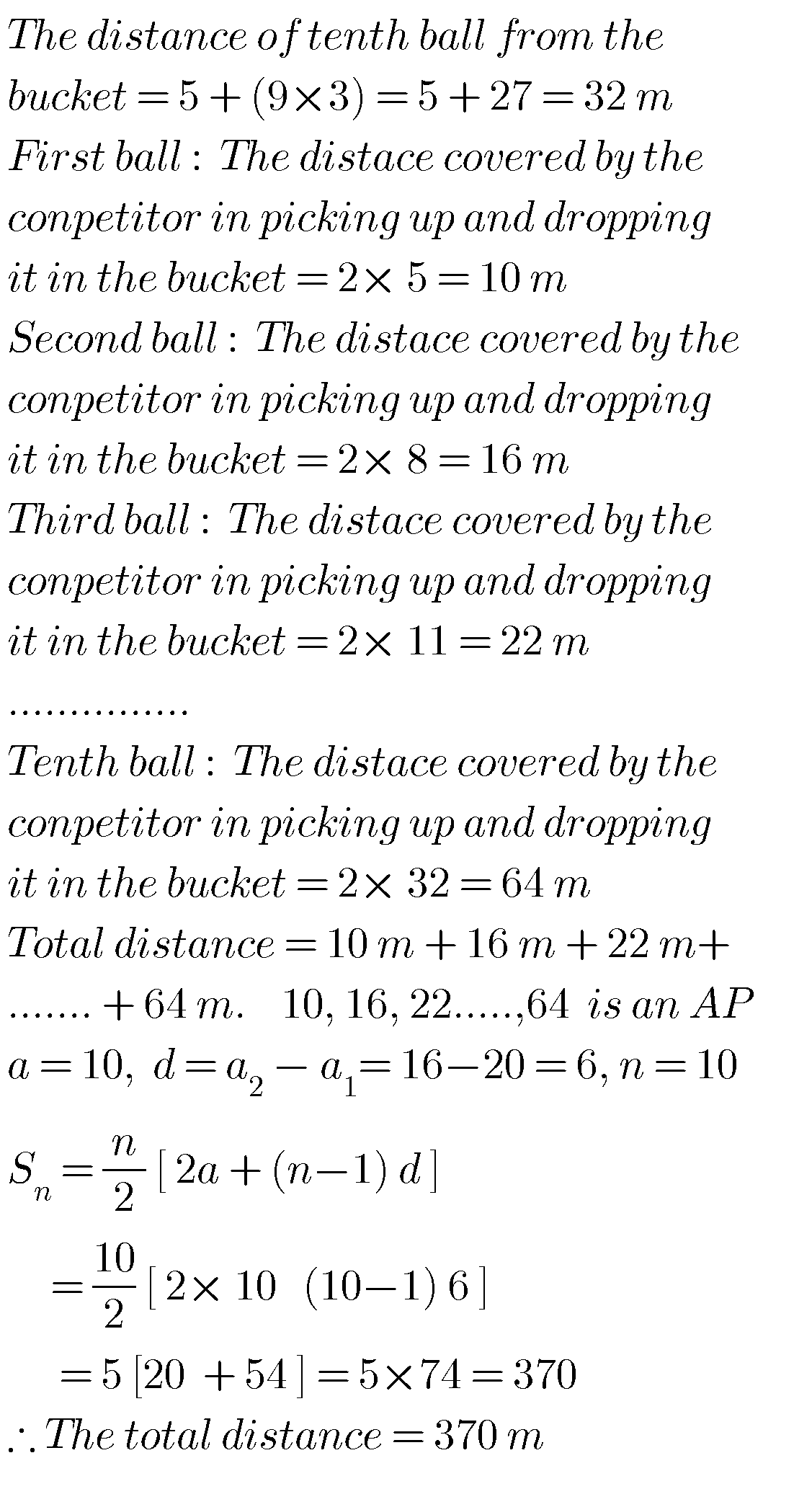### Chapter 6 Progressions solutions class 10 ssc

Exercise 6.4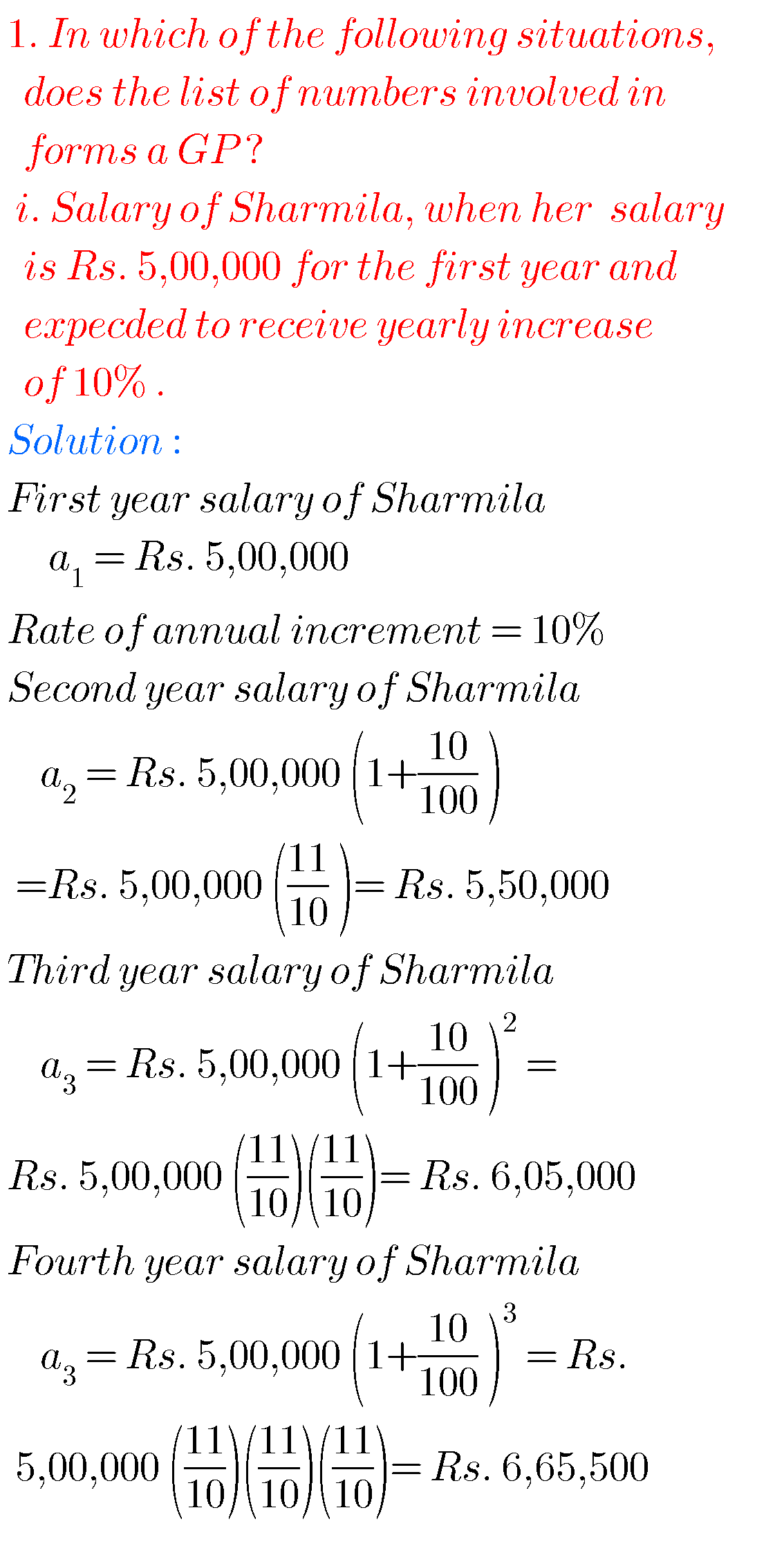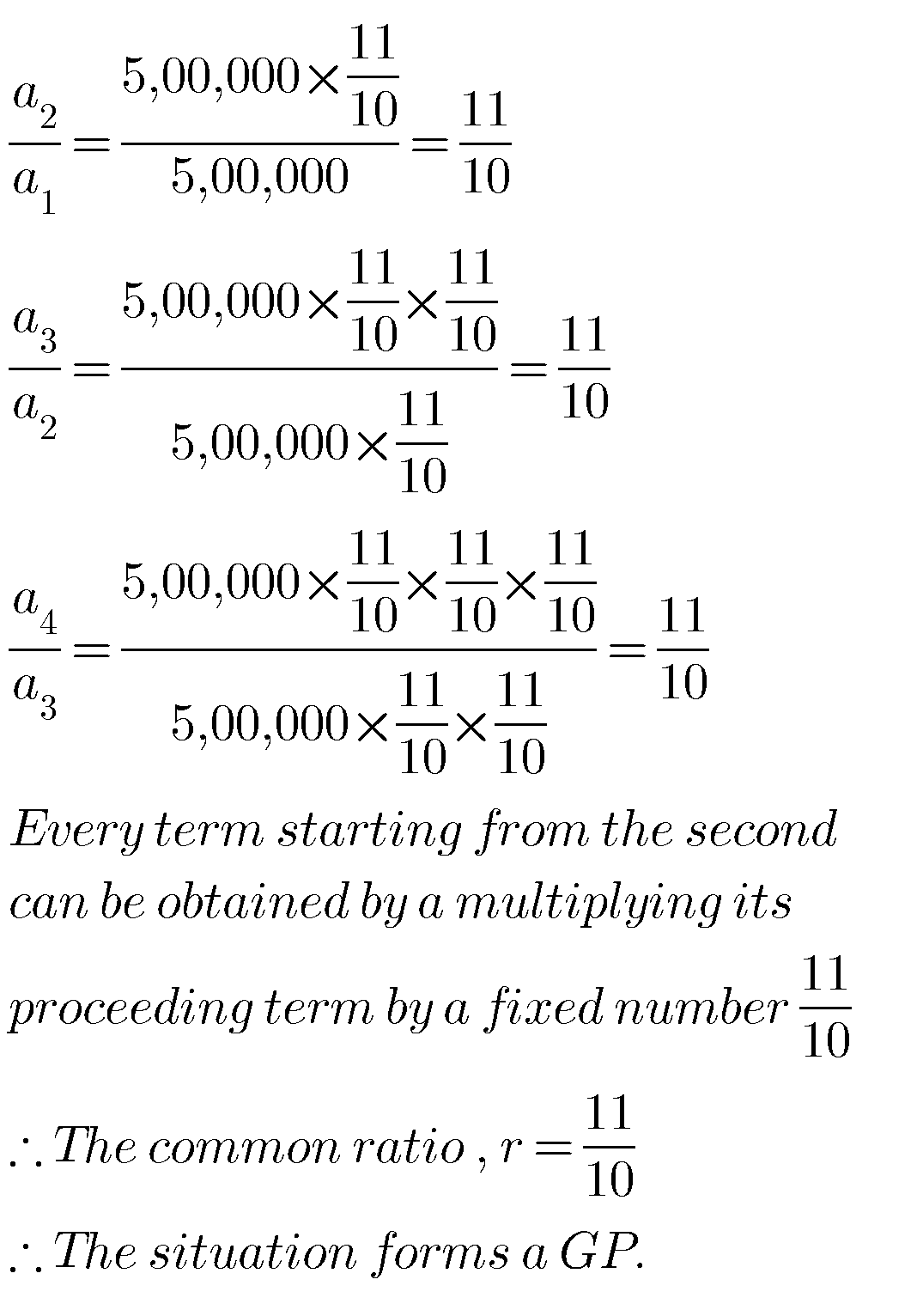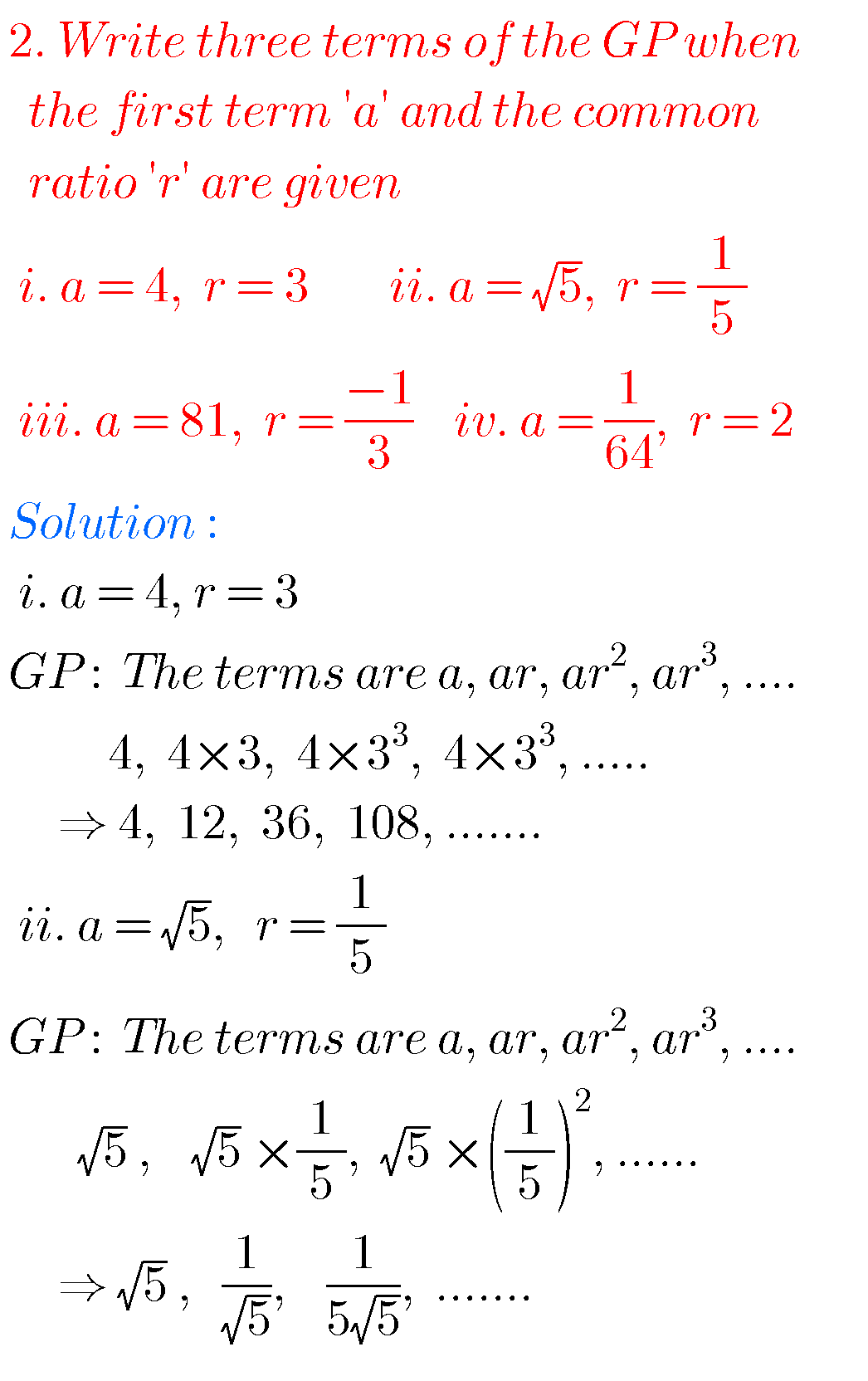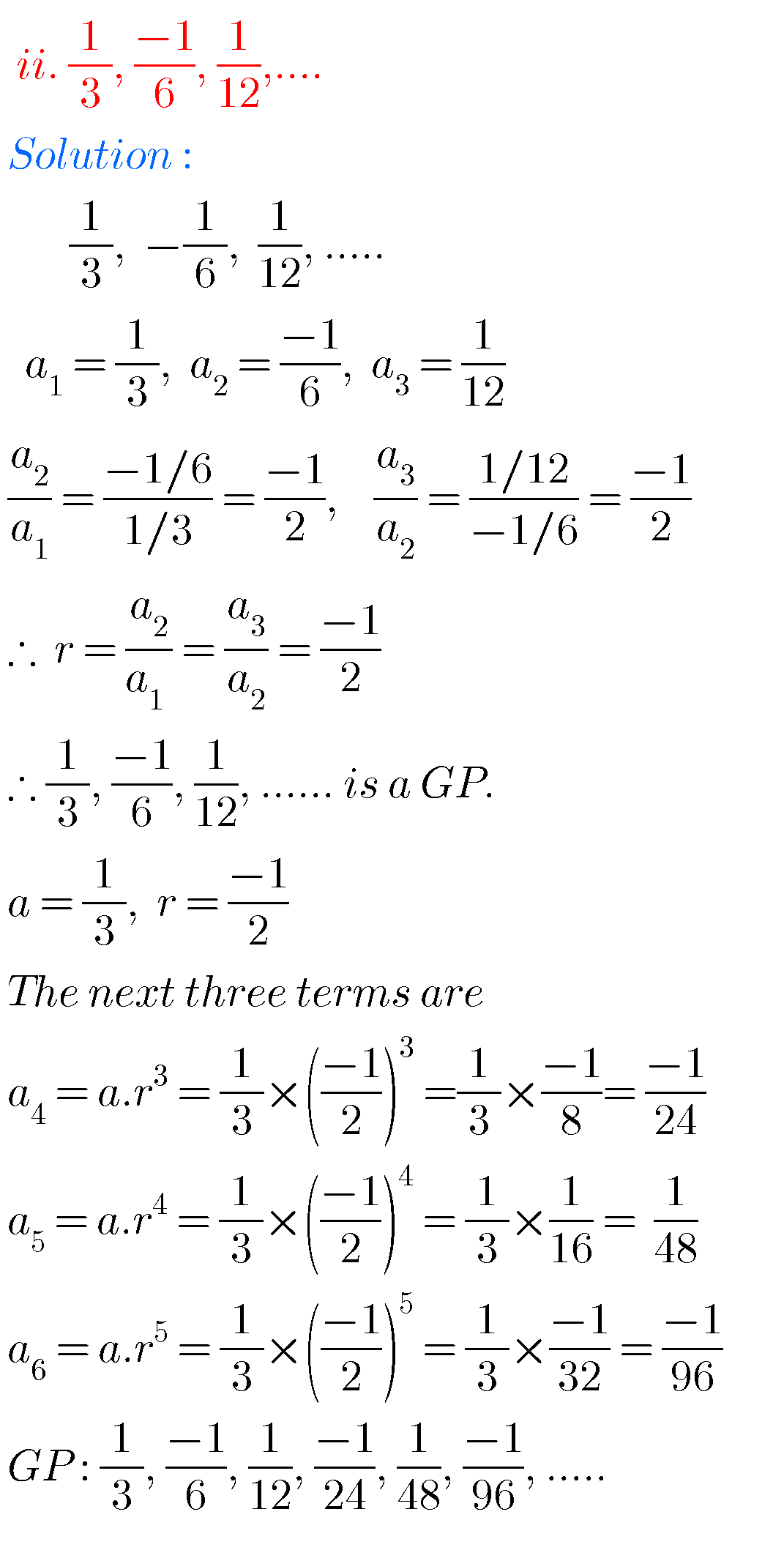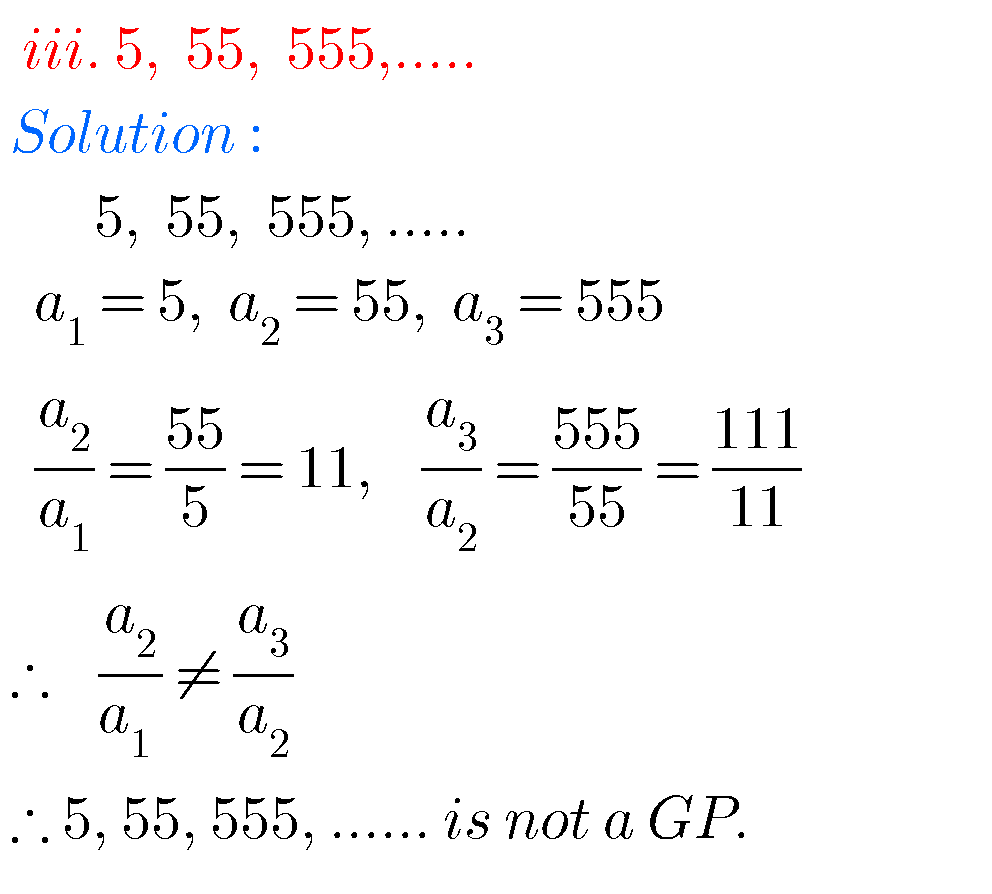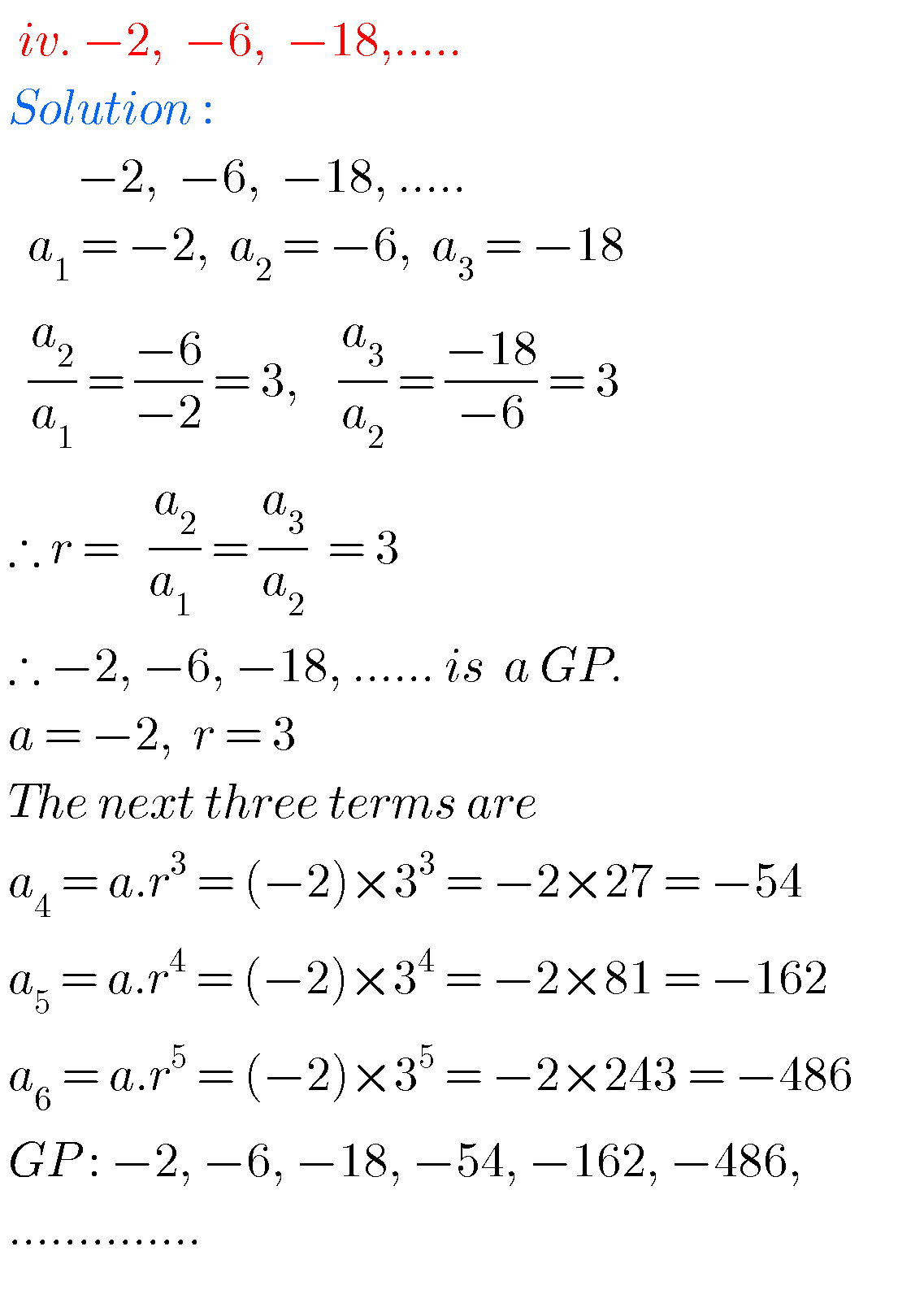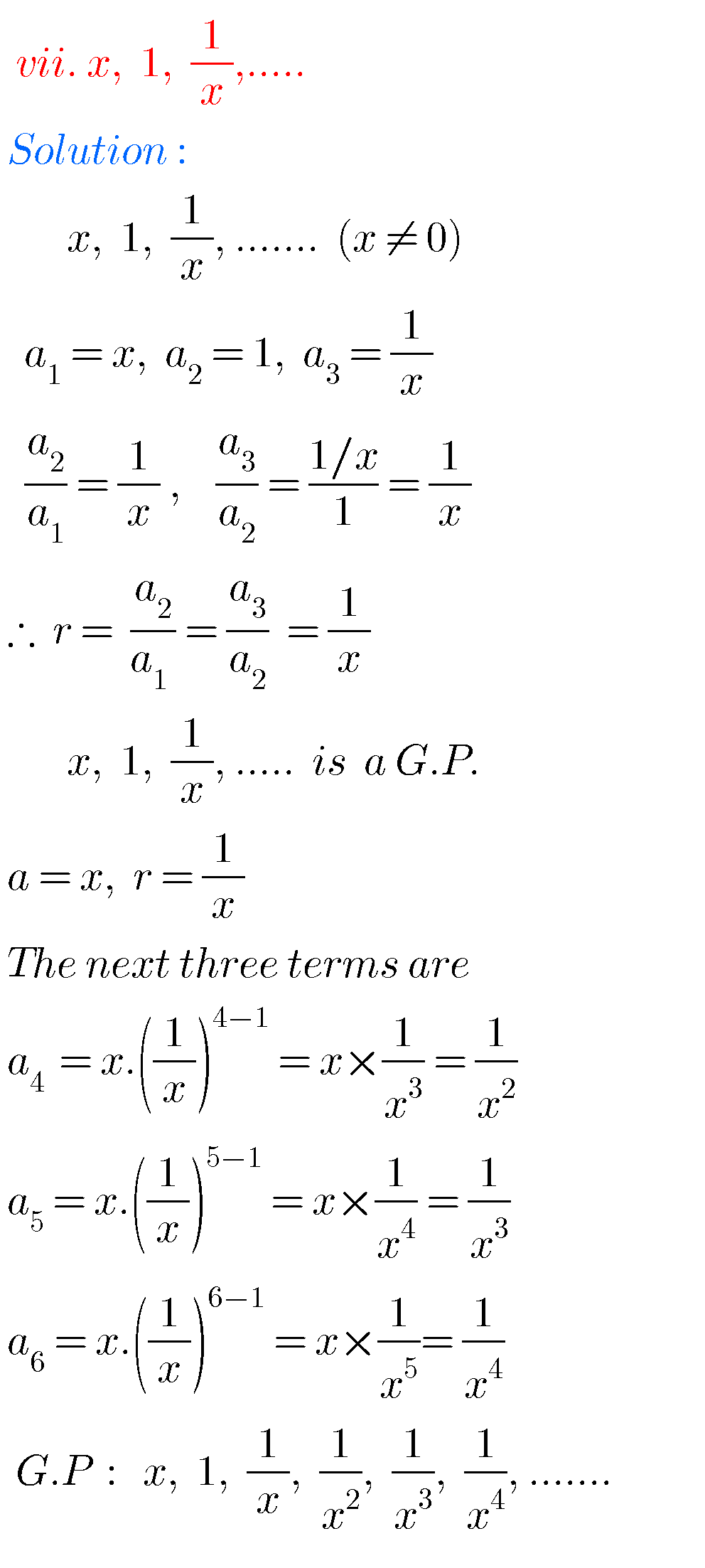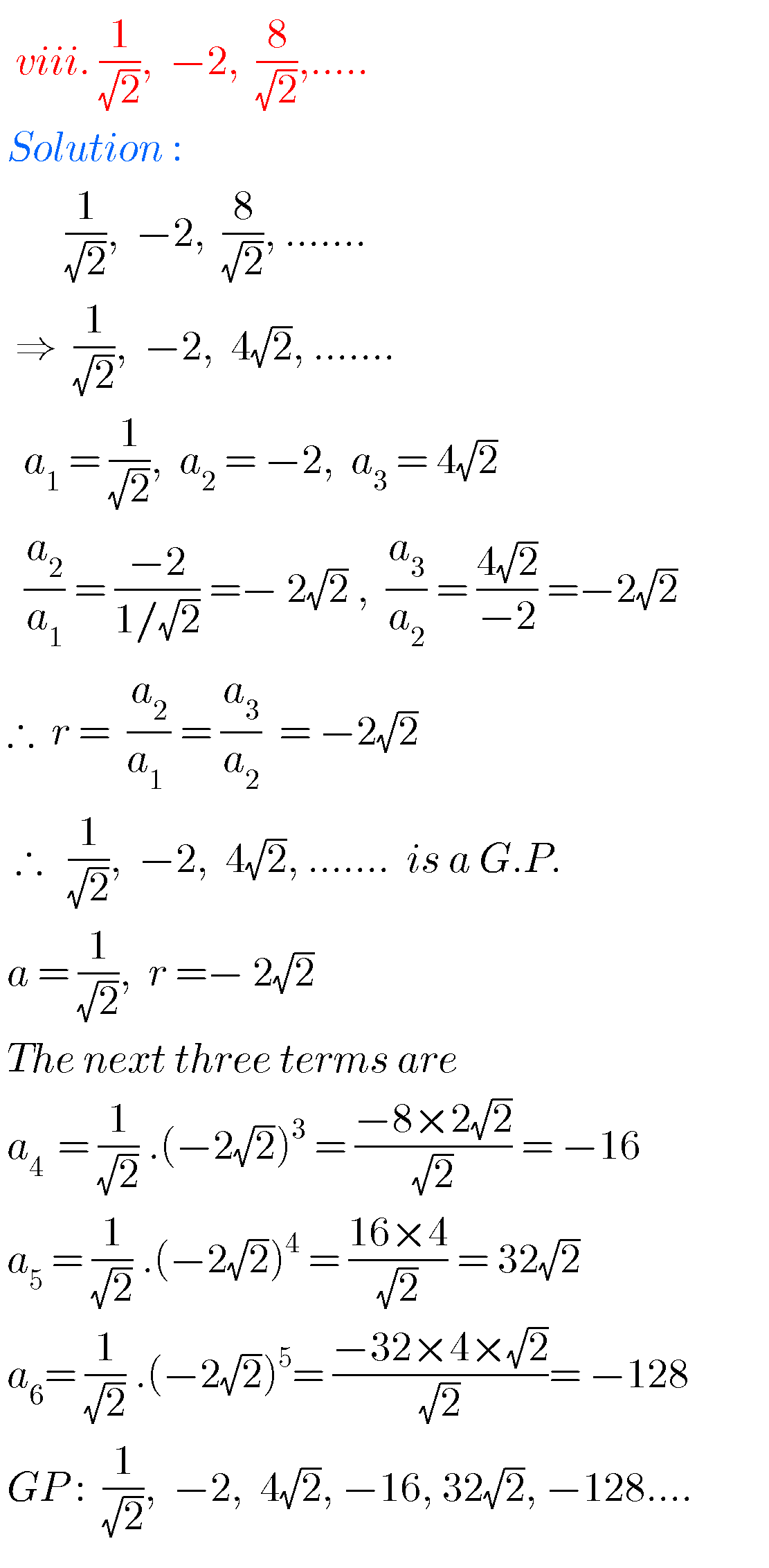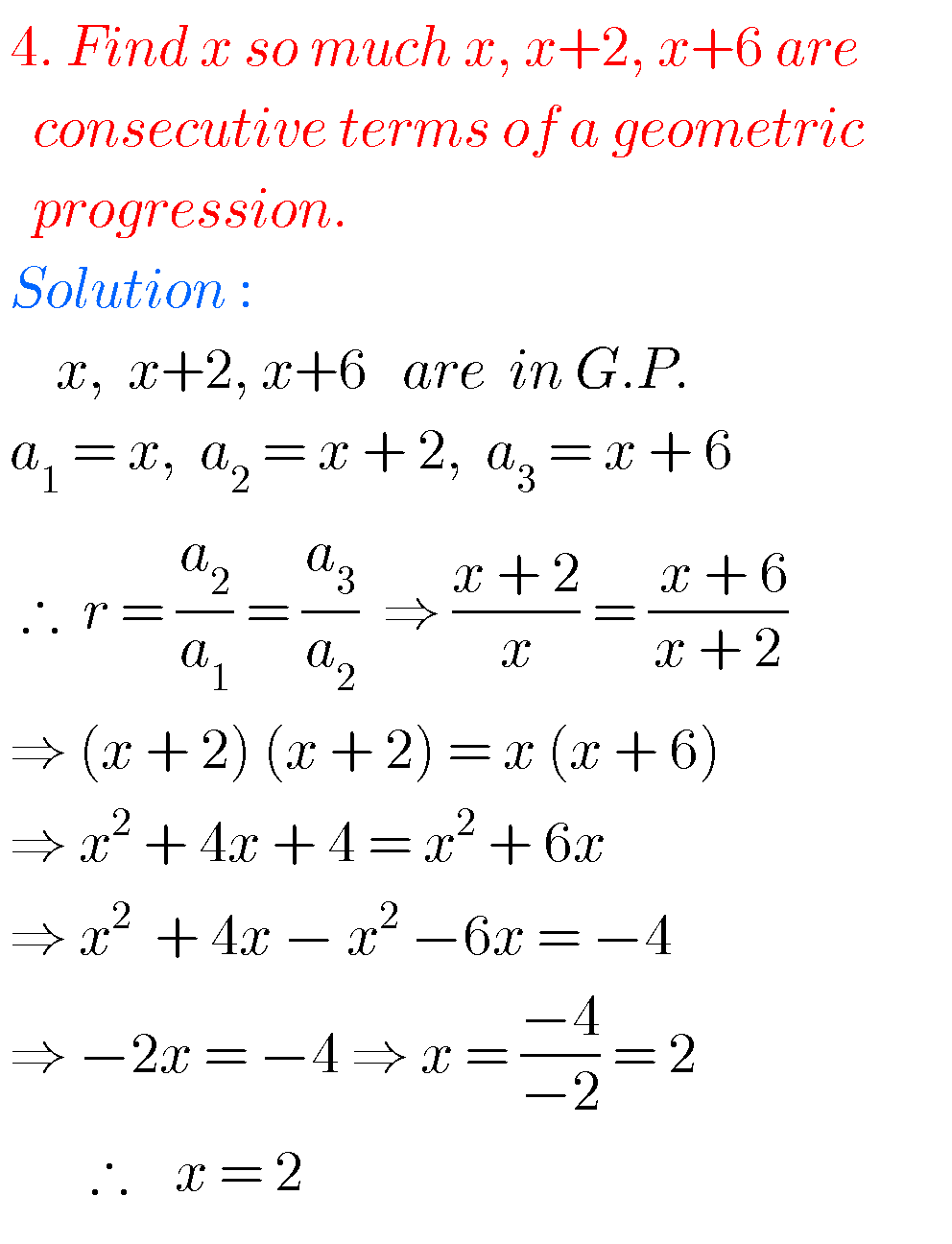### Solutions for Progressions class 10 SSC

Exercise 6.5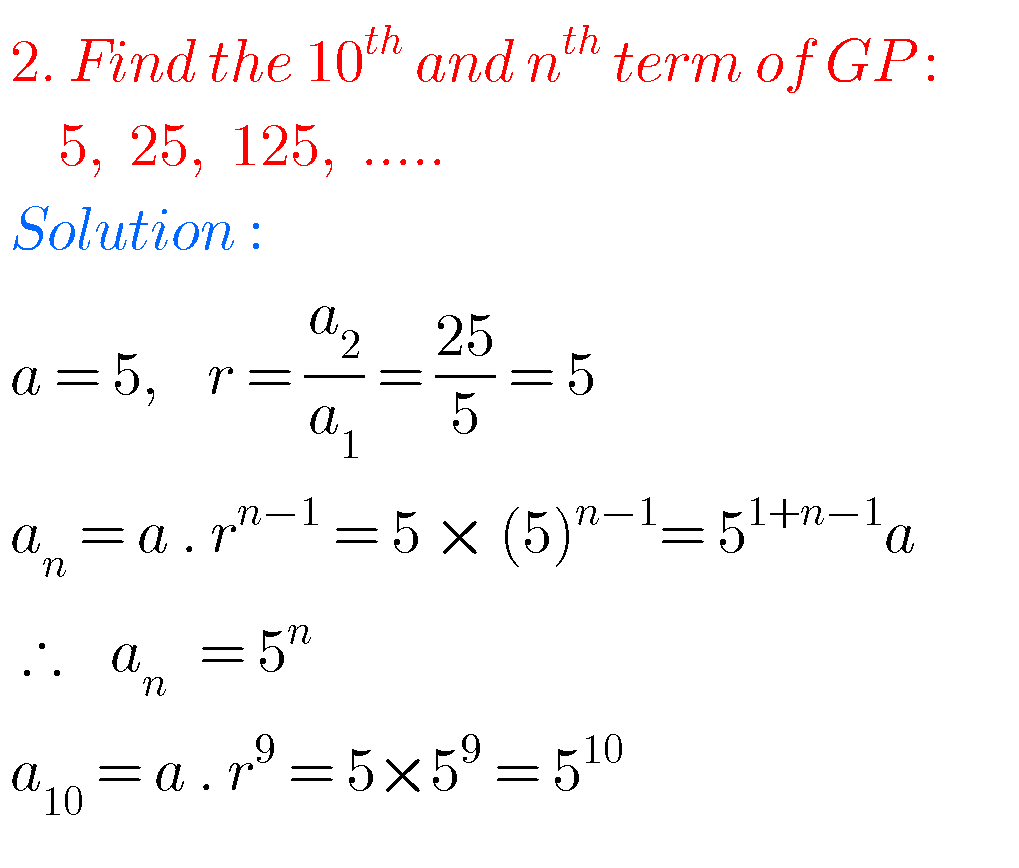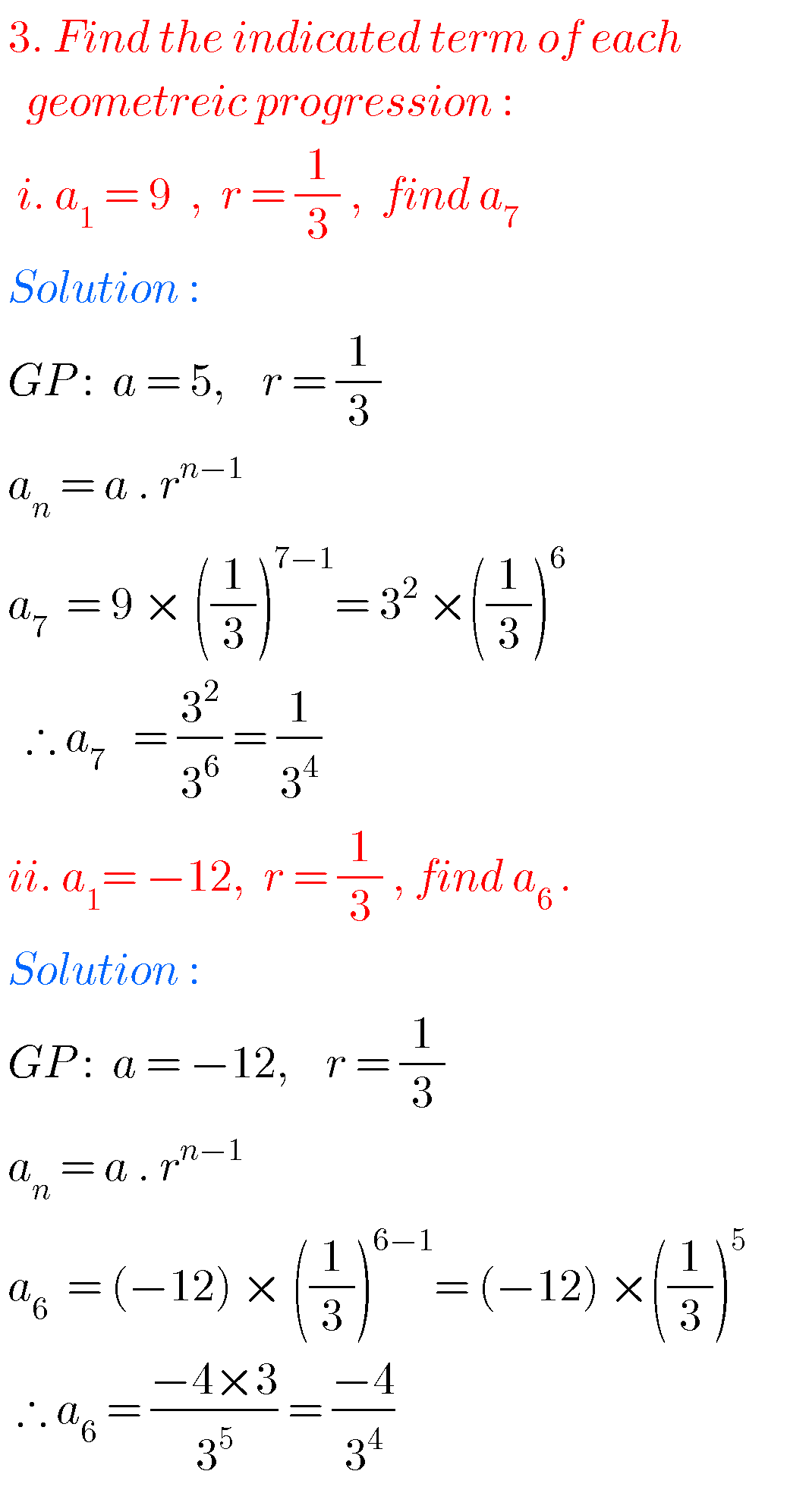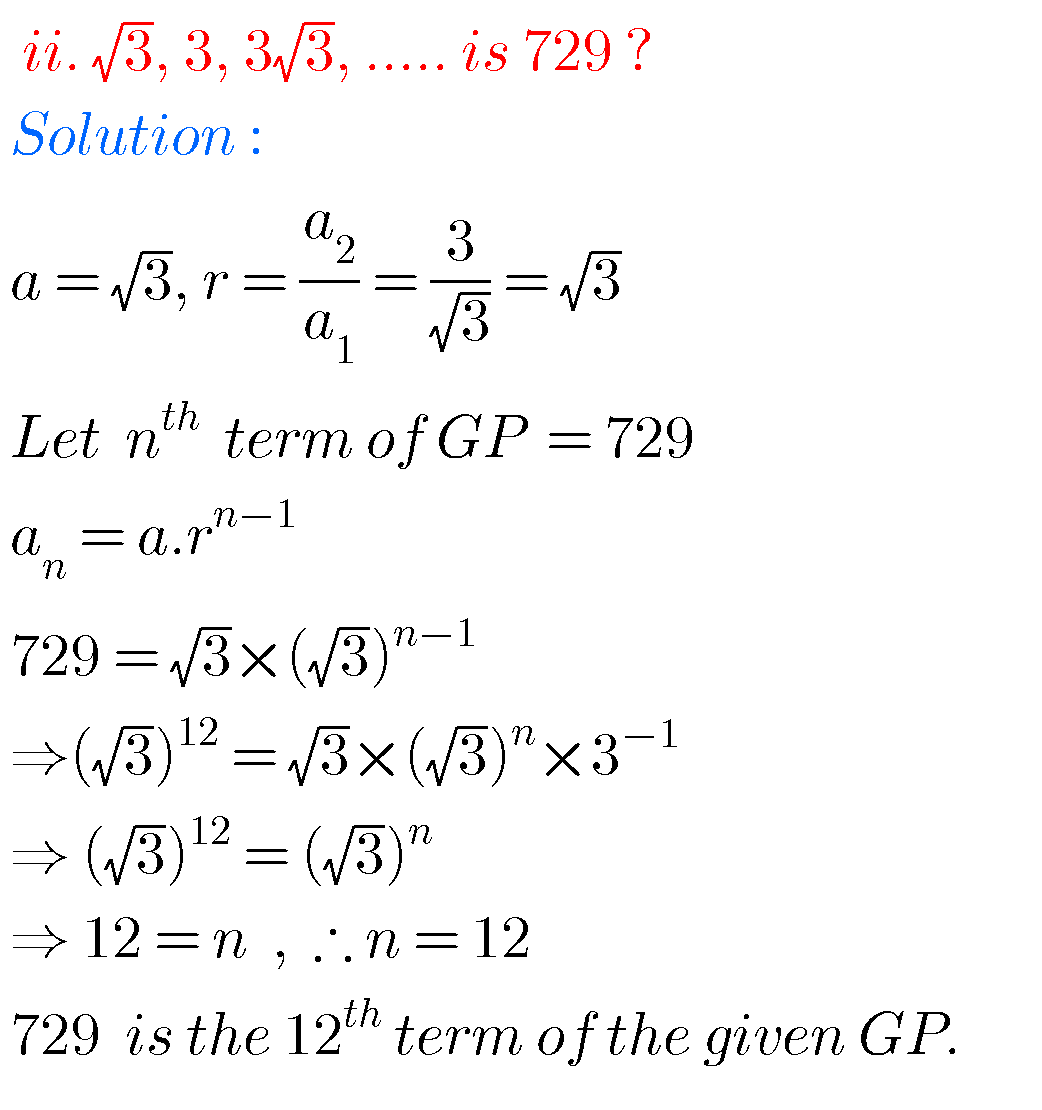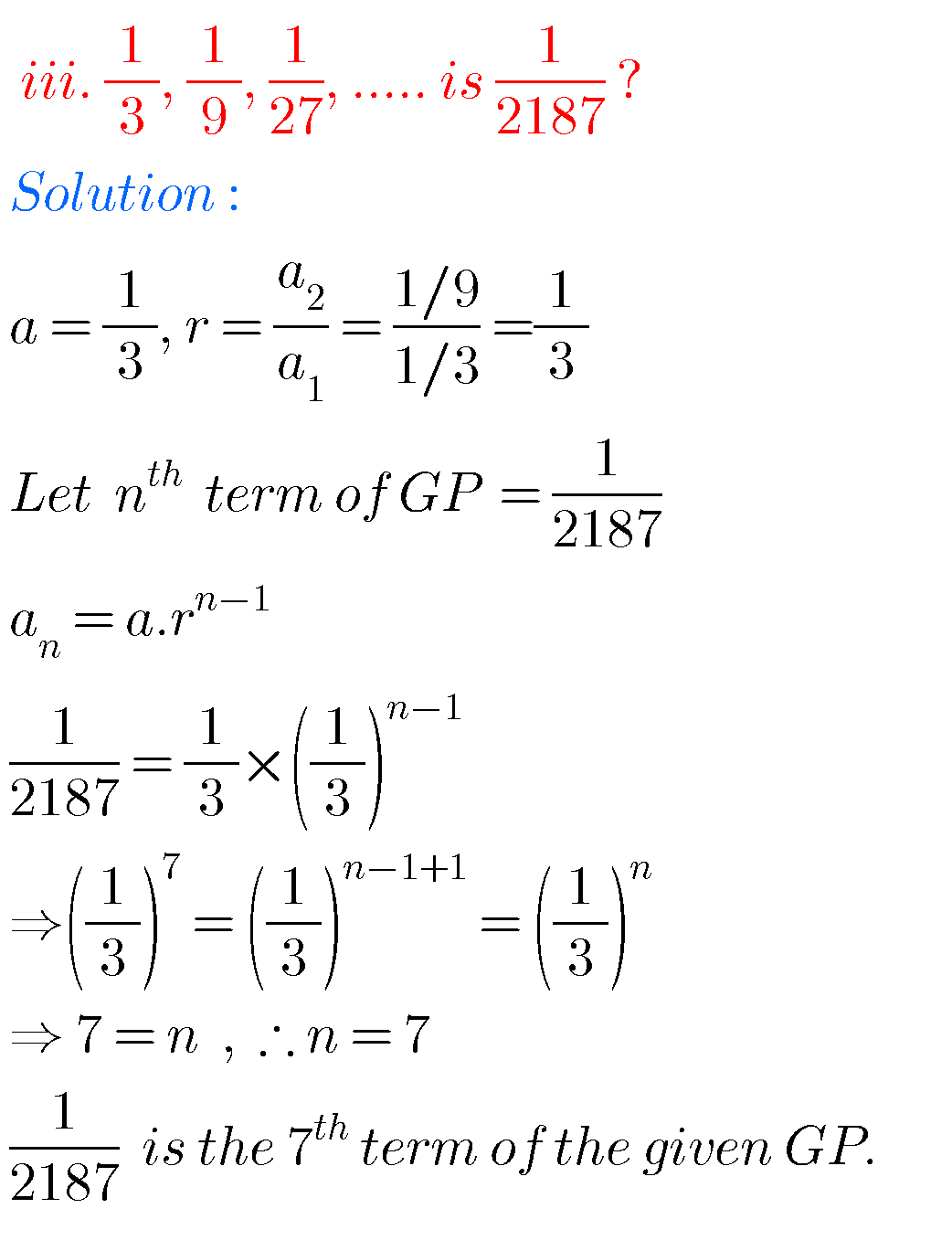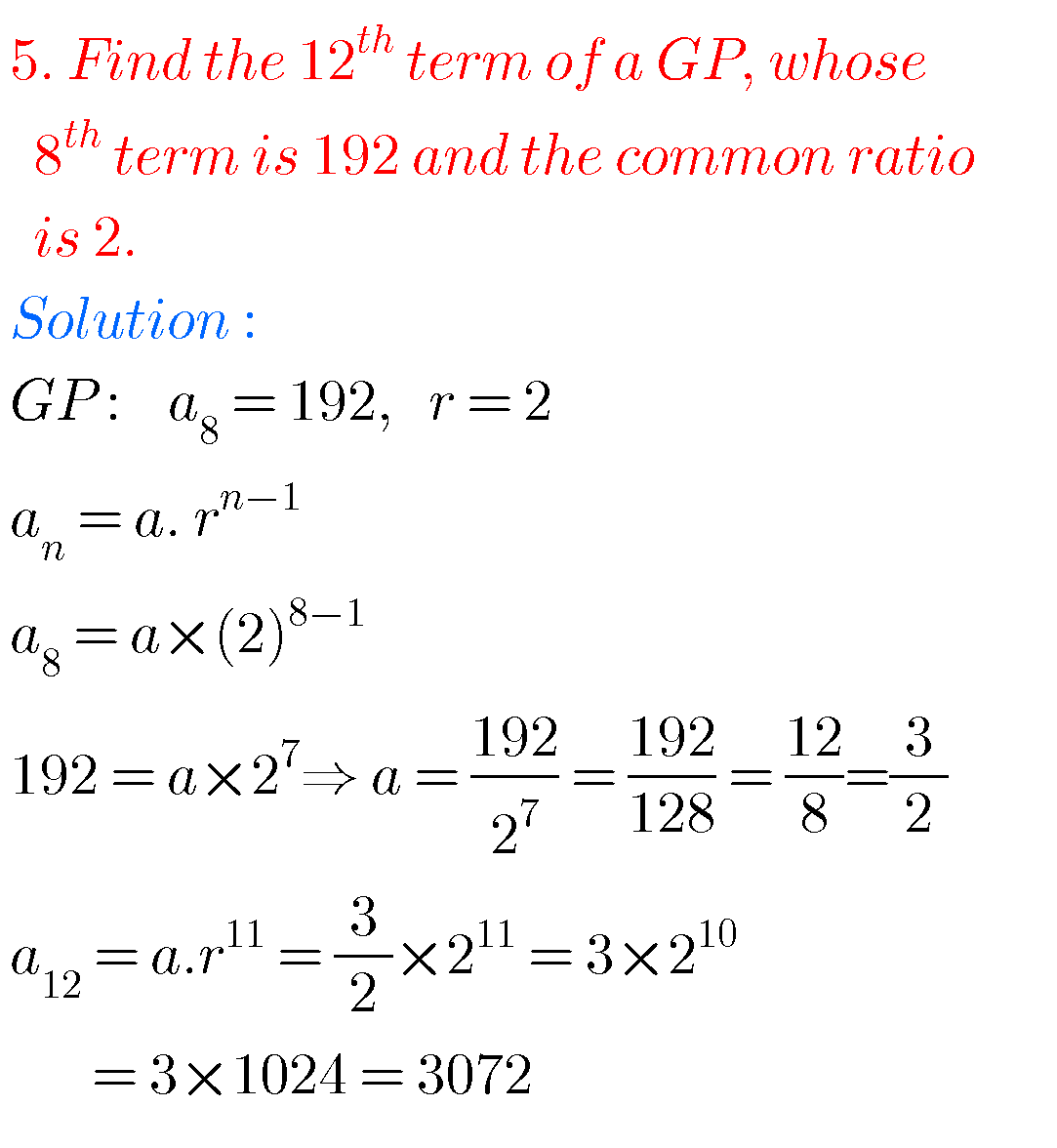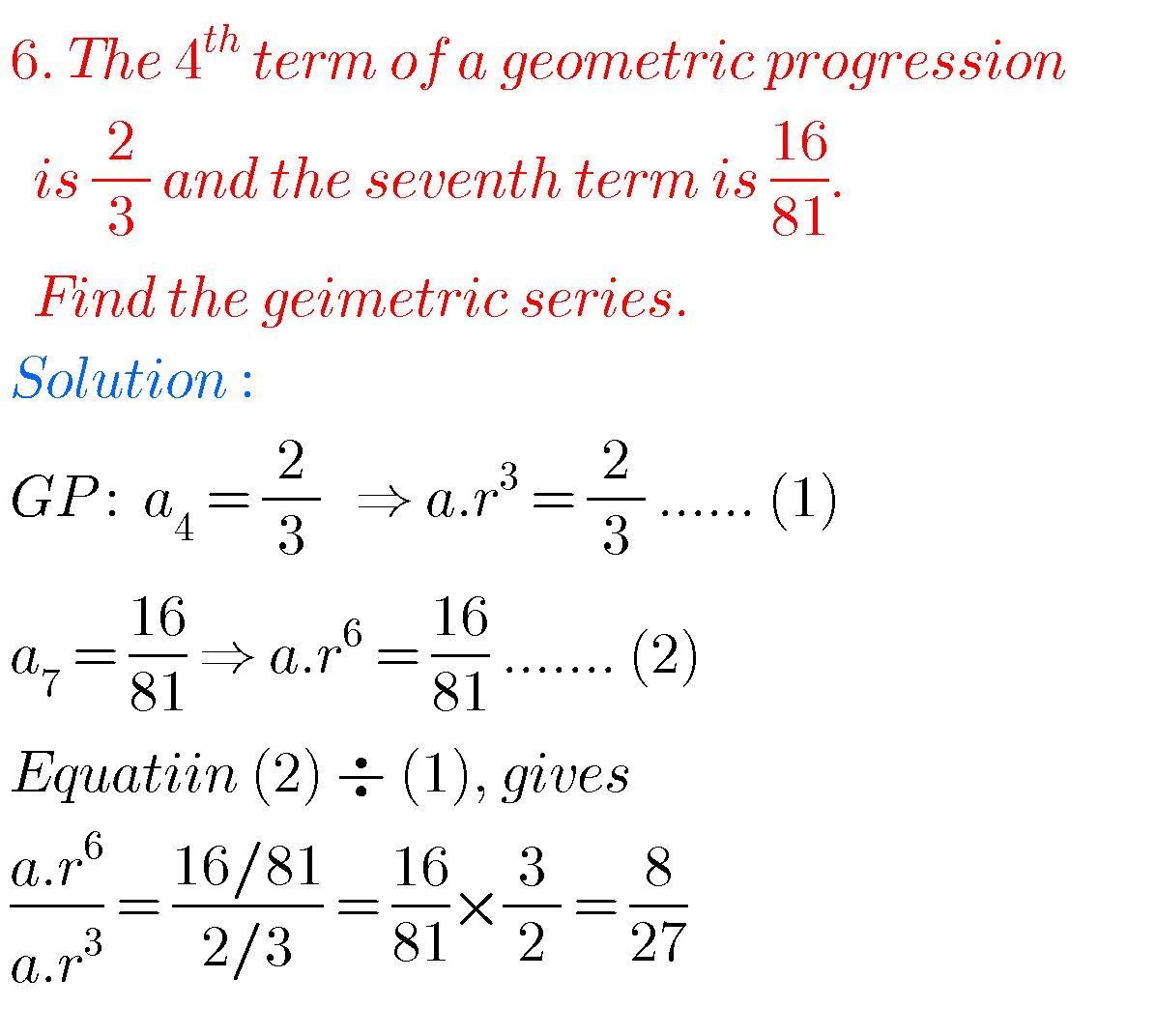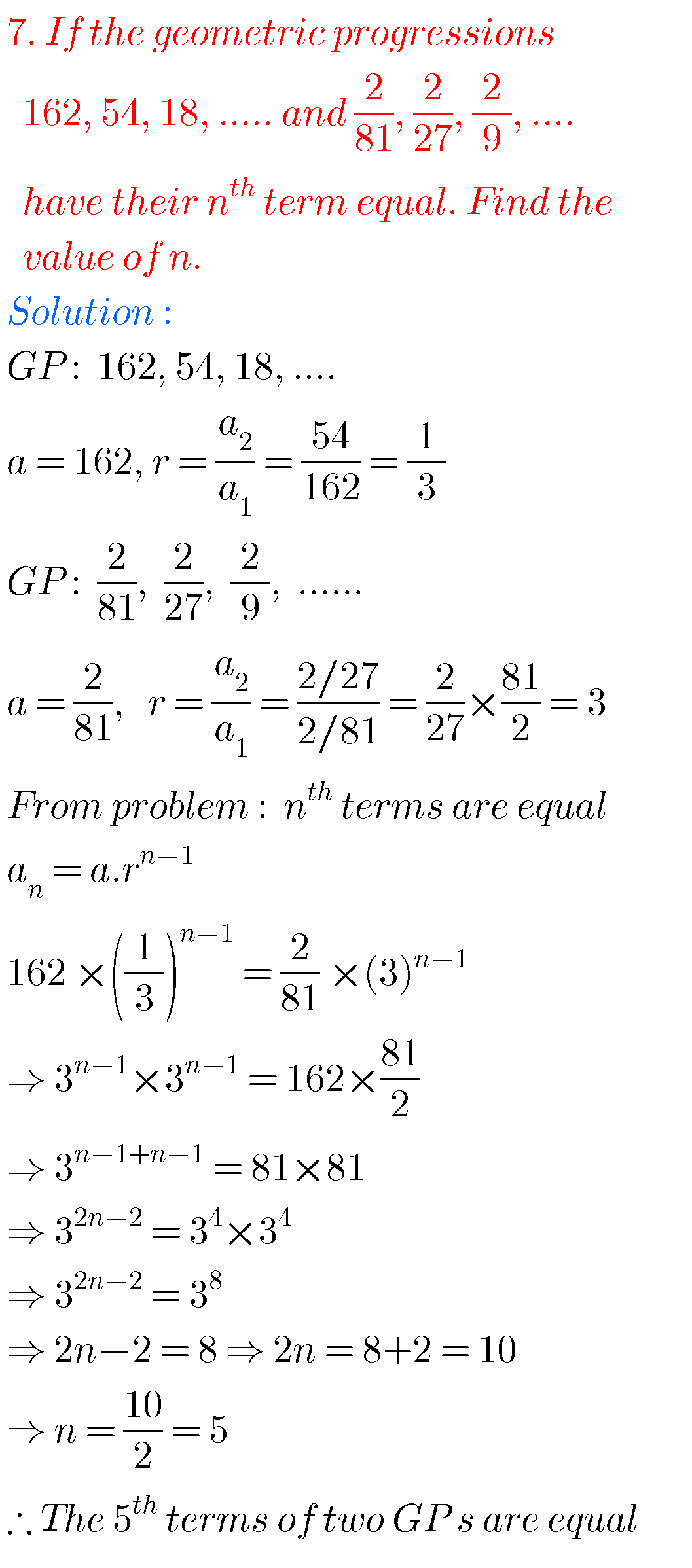Note : Observe the solutions and try them in your own method.

Inter 1a exercise 6(a) solutions

Inter maths 1a exercise 6(b) solutions

Ncert maths class 8 exponents and powers

Ncert maths class 7 fractions and decimals

Ncert maths class 6 playing with numbers

Ncert solutions for maths class 7 rational numbers

Ncert solutions for maths class 6 knowing our numbers

Ncert solutions for maths class 6 integers

Ncert solutions for maths class 7 integers

Ncert solutions for maths class 6 whole numbers

You can see solutions for Inter Maths IIB

1. Circle

3. Parabola

4. Ellipse

You can also see solutions for Inter Maths IIA

For examination purpose you can see

Complex numbers

De Moivre’ s Theorem

### One Comment

1.Deepika November 27, 2020 at 9:43 am - Reply

Superb yaar